# Credit Card Fraud Detection - Part 1

Temps de lecture : 7 min

Publié : janvier 8, 2023

Mis à jour : janvier 8, 2023

### Format video

How do we know when a credit card is fraudulent or not?

This is a classification problem statement.

This tutorial will be in 2 parts, the first part will be on machine learning, and the second will be on deployment.

I like typing the problem statement, so let’s write in markdown what we’ve said before, whether this credit card is fraudulent or not. Now, about the dataset. We can find it on Kaggle. There is some information about it. Class 0, 1. the statistics. You can download the dataset here to get the archive. We type the link in our notebook.

https://www.kaggle.com/datasets/yashpaloswal/fraud-detection-credit-card

Don’t forget to you put the CSV in your folder.

To get it, just click on download, then unzip the archive to get this CSV file.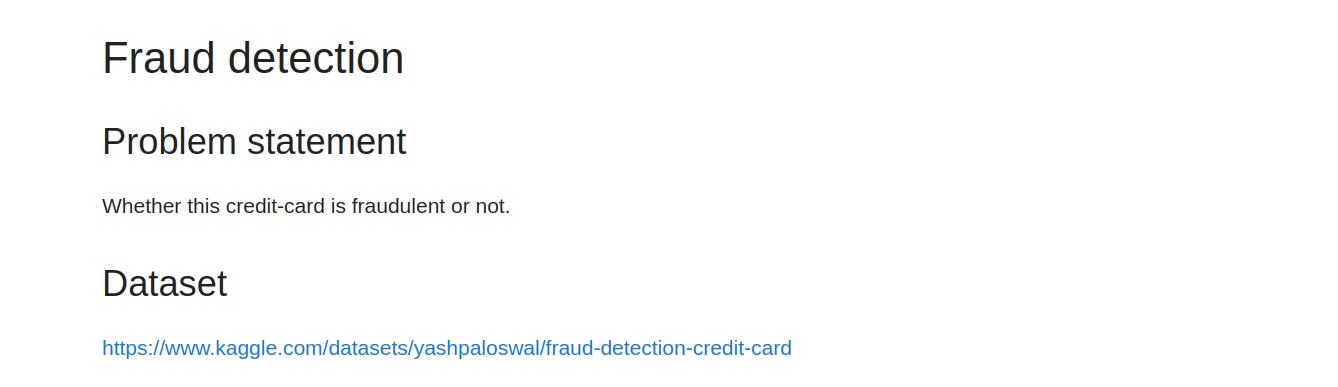### 1. Importing Libraries

We import numpy as np (for algebraic operations on arrays), pandas as pd (for data exploration and manipulation). Then matplotlib, seaborn plotting libraries. The inline is a magic function for visualisation.

1import numpy as np
2import pandas as pd
3#for algebraic operations on arrays
4#for data exploration and manipulation
5from sklearn.preprocessing import StandardScaler
6
7# plotting libraries
8import matplotlib.pyplot as plt
9import seaborn as sns
10%matplotlib inline

We load the dataset. We create a variable named train with the file path, and create a new variables named df_train to create a dataframe.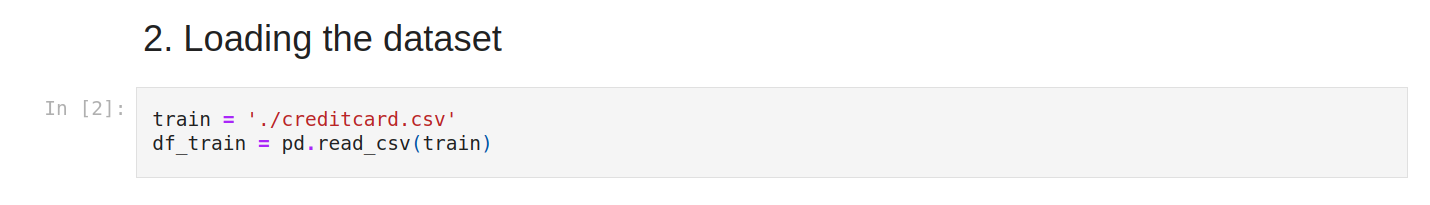### 3. Exploratory data analysis

Let’s view the first five lines. For that, we use .head(). at first glance, we see numerical values. We don’t understand the data. so it seems to be changed with PCA. It’s normal because credit card data needs to be confidential.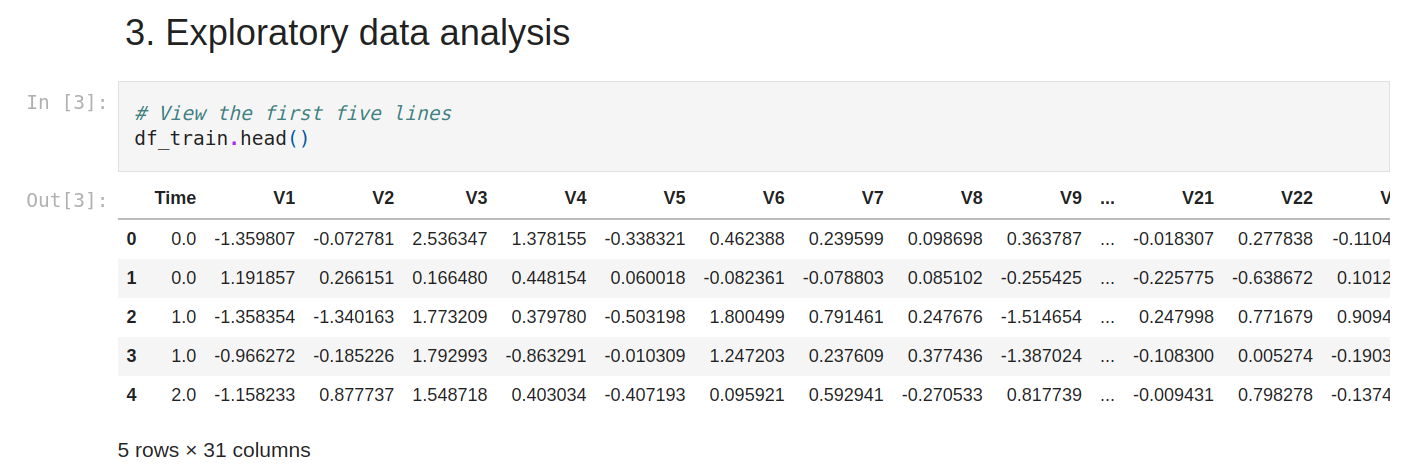Now let’s see the shape., A lot of lines.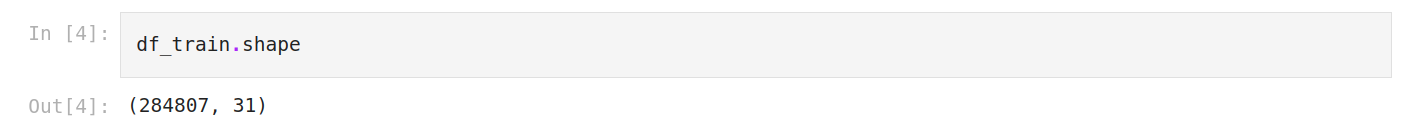We check the data information. It will help us to to interpret a little bit. So we have 284807 rows, 31 columns, all numerical features and no missing columns.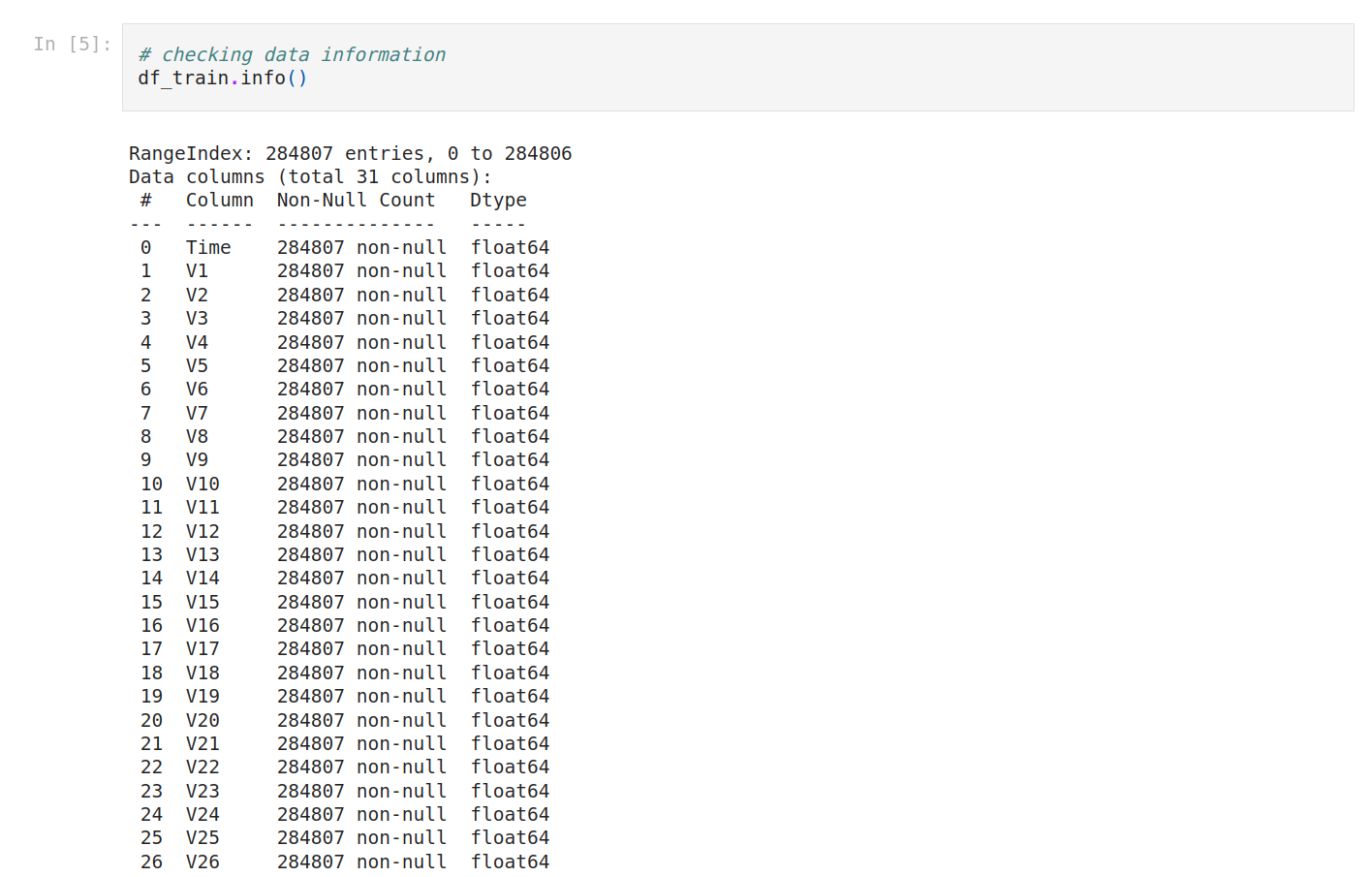Let’s type that.

Interpreting Data Information

• We have 284807 rows, any column that contains a lesser number of rows has missing values.
• We have 31 columns.
• There are numerical features that have data type float64.
• There are numerical features that have data type int64.

No missing columns.”

The columns’ names are a little bit different, so let’s normalize everything in lowercase.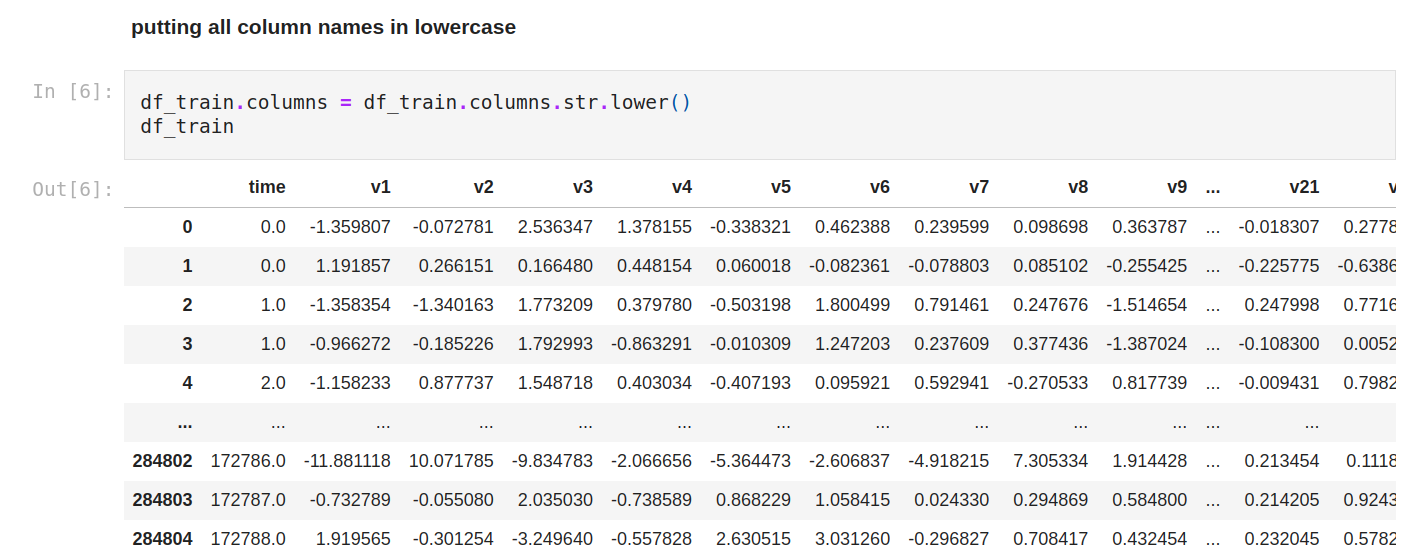Let’s see the statistics of numerical variables (so every variable).

We can compare the mean of each column with the min/max value, to check if we might have outliers as there's a considerable difference between the average value and the max value.

We can compare the general mean and standard deviation to see if we need to normalize the data as there's a considerable difference between all of them.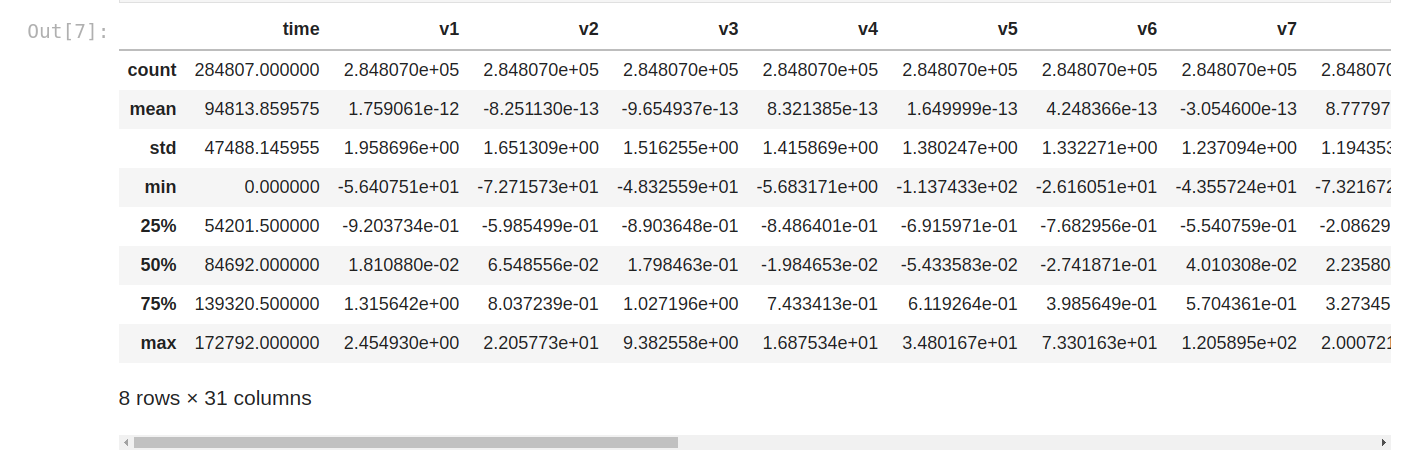Now, we’re gonna go with univariate analysis.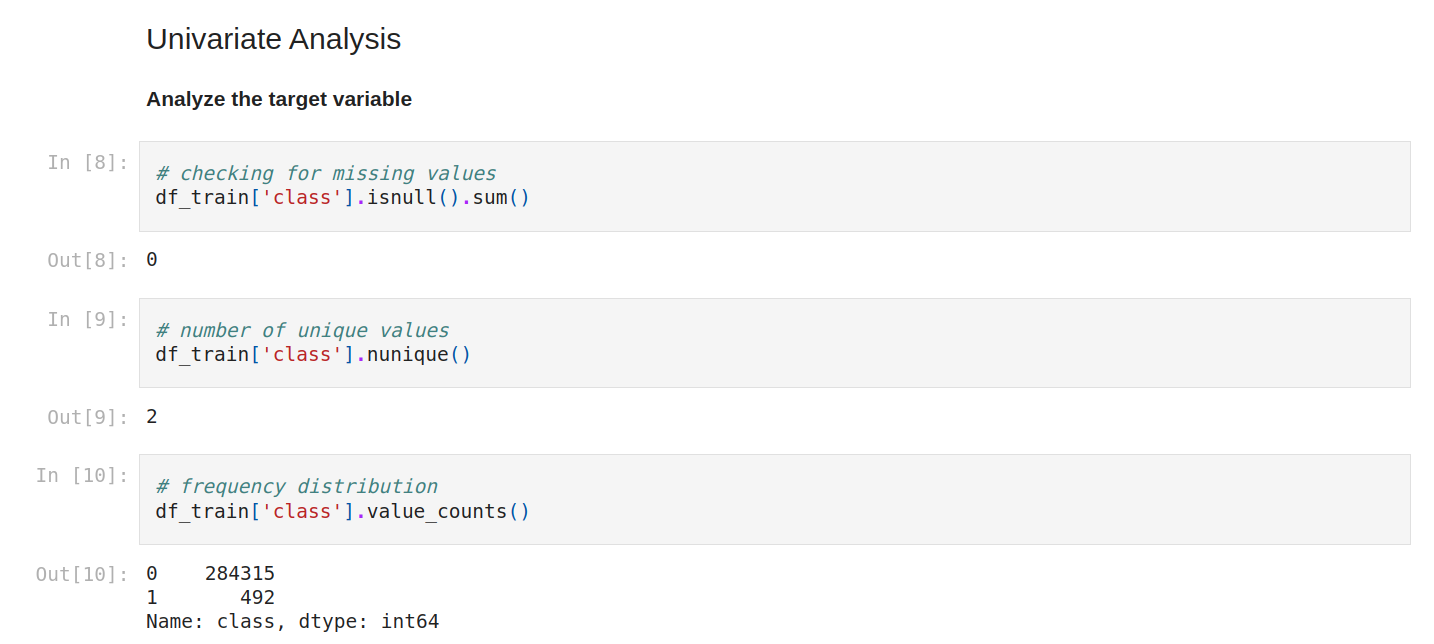For numerical continue variables, we can use a histogram or scatter plot, for categorical data, we commonly prefer bar plots or pie charts.

The goal is to analyze the target variable.

We can check again for missing values. Yes, still zéro! We can check the number of unique values and the frequency distribution.The percent breakdown of the target. As you can see, this is the same code as the frequency distribution, but we normalize it as true.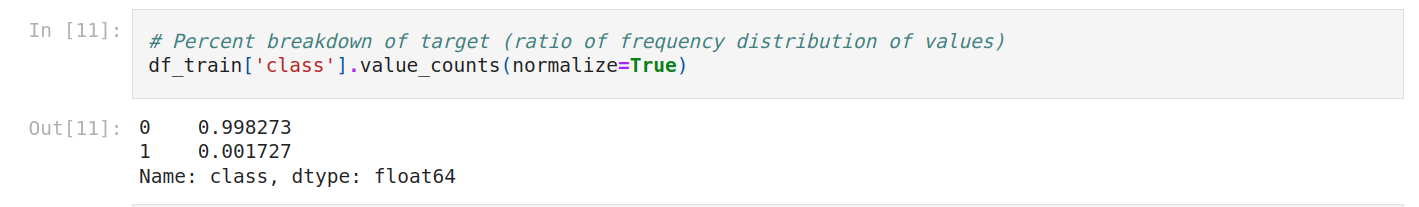I think it would be better to plot it, so let’s do that.

The dataset is very imbalanced (99/1) we can't use accuracy because of the huge imbalance.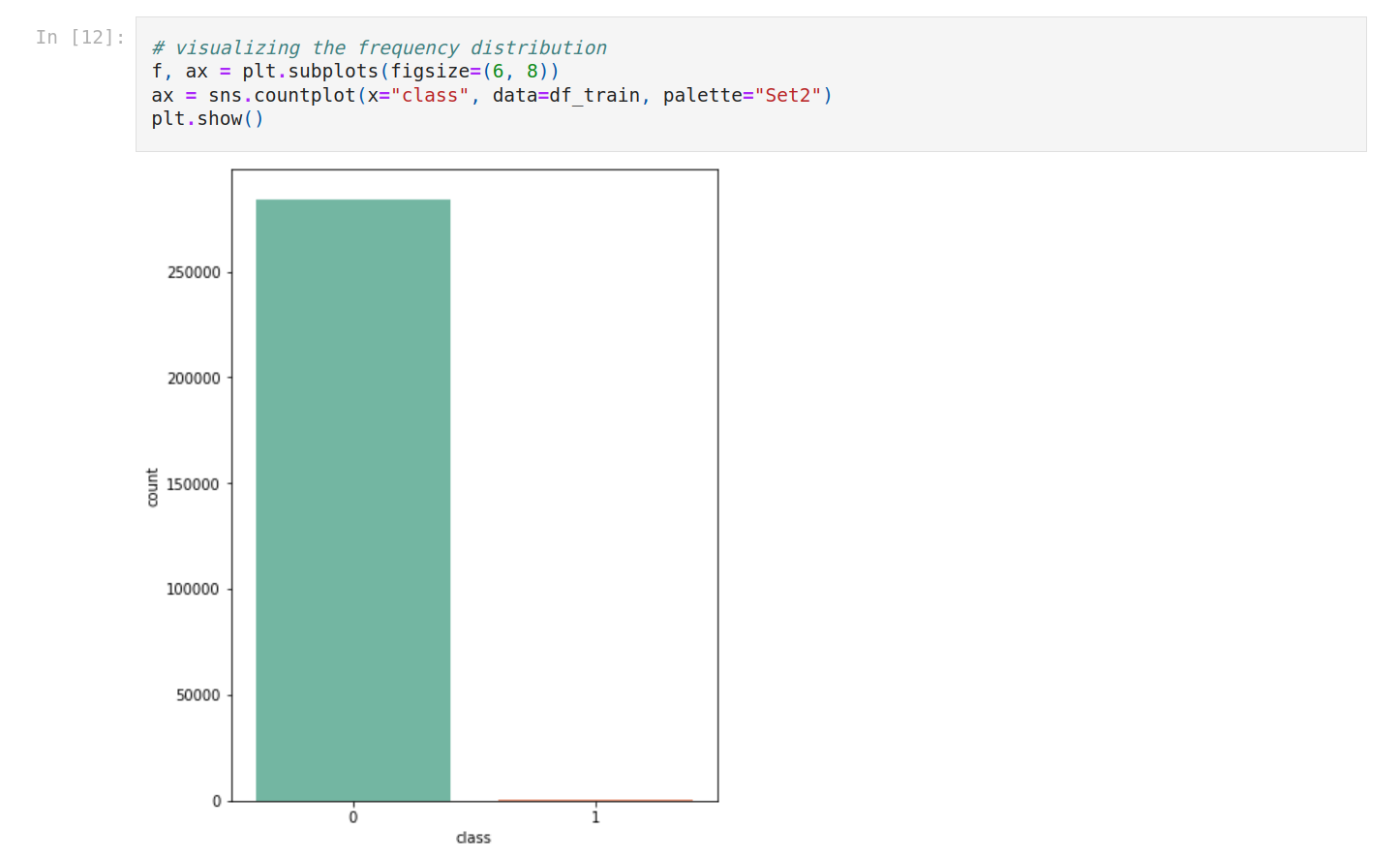Accuracy is correct predictions on all predictions. Super simple.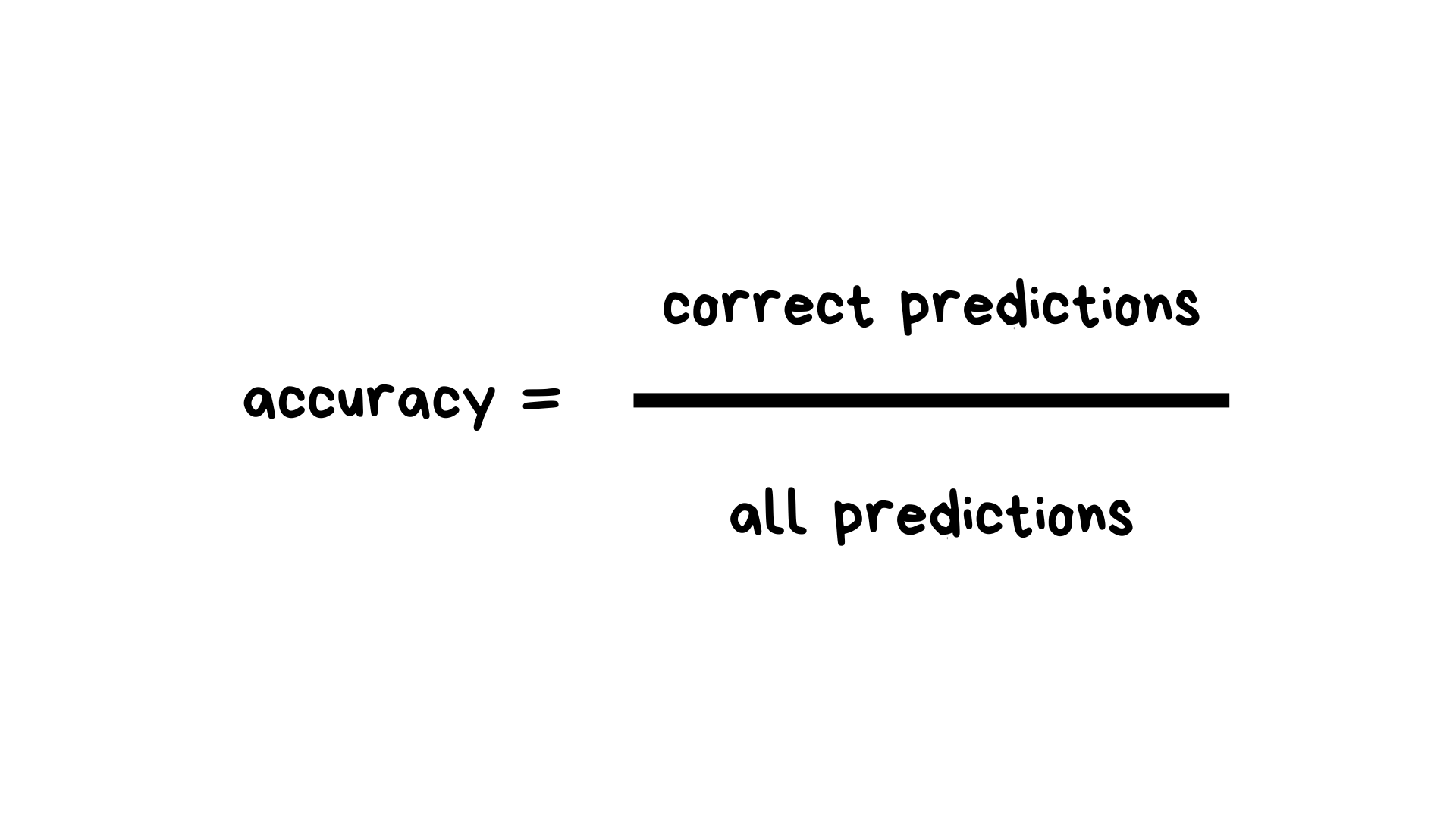This is a balanced dataset.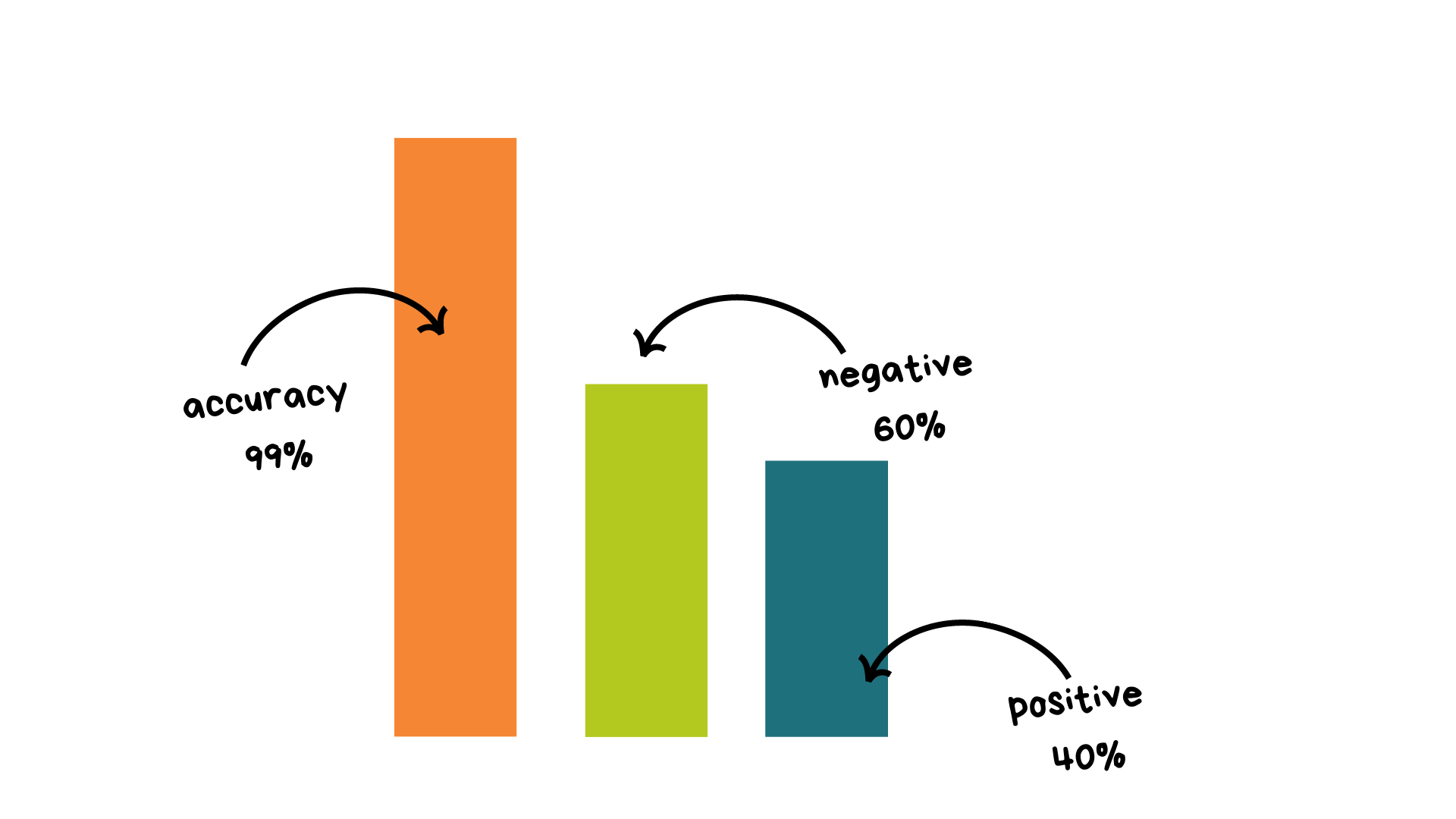The thing is, we want to create a baseline model to have a minimum score to beat after with better models. If we have 99% of negative values, our score will be around this number, and how can we beat that? This is too high.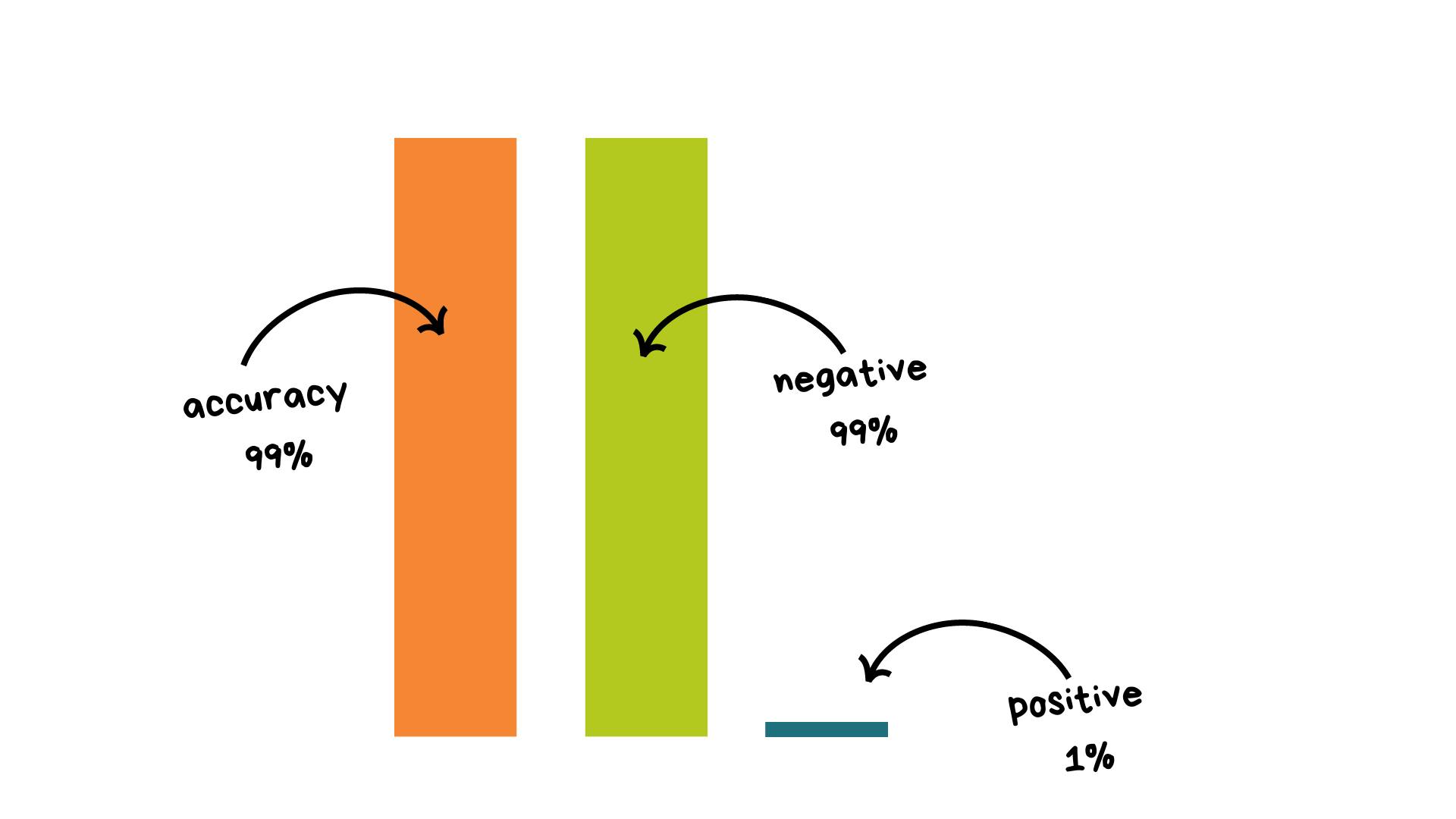So, we have positive and negative values, and I think you know true positives, false positives….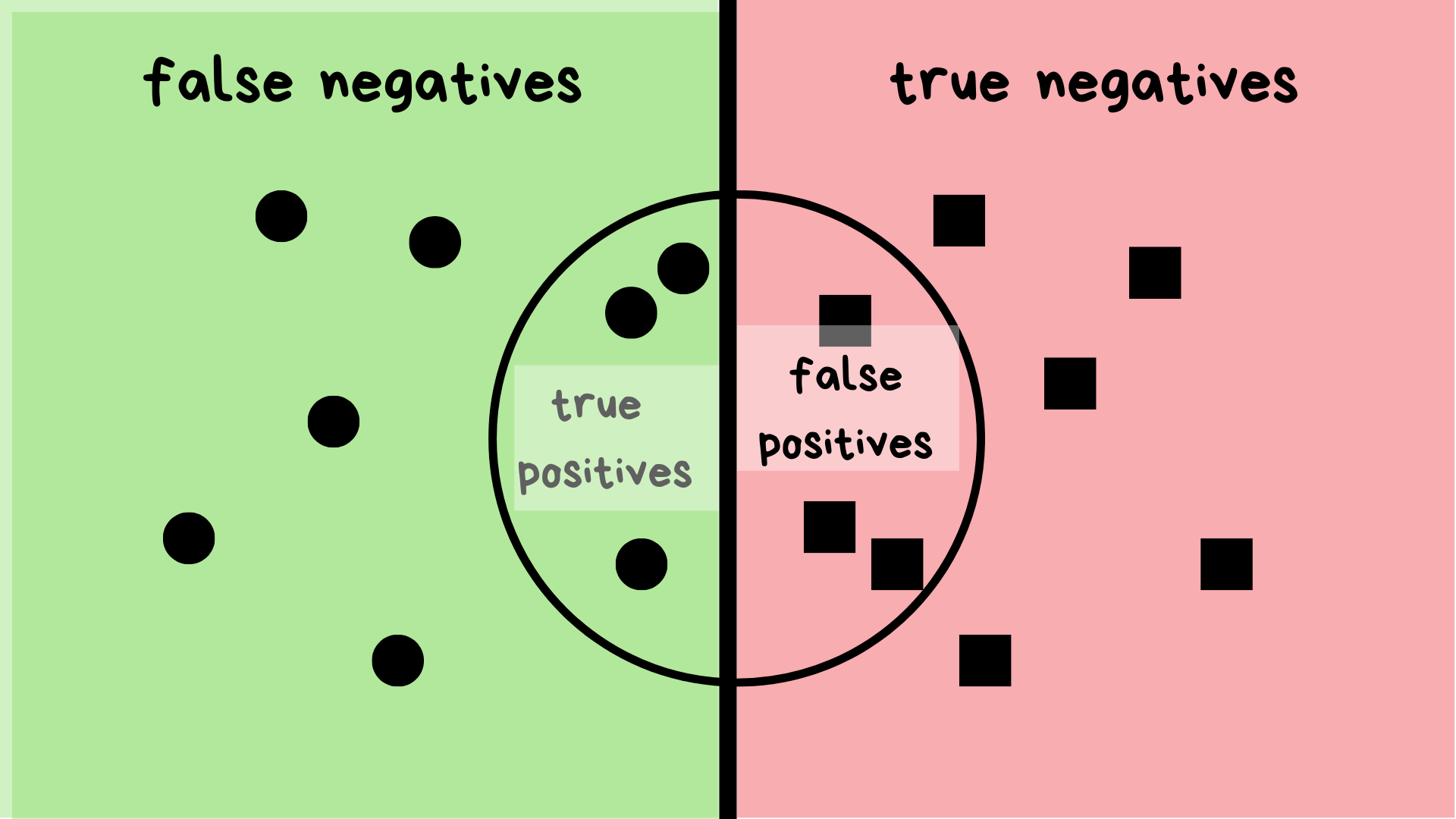We can type how many cards are fraudulent.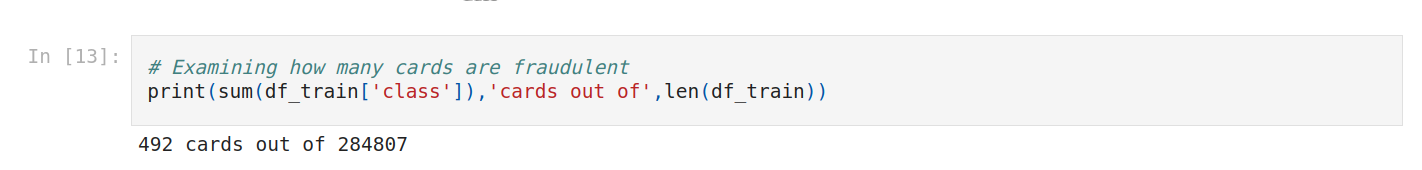Bivariate Analysis requires you to learn about relationships between pairs of variables. We can use a scatter plot, a pair plot, or a correlation matrix.

We can for example compare amount with class.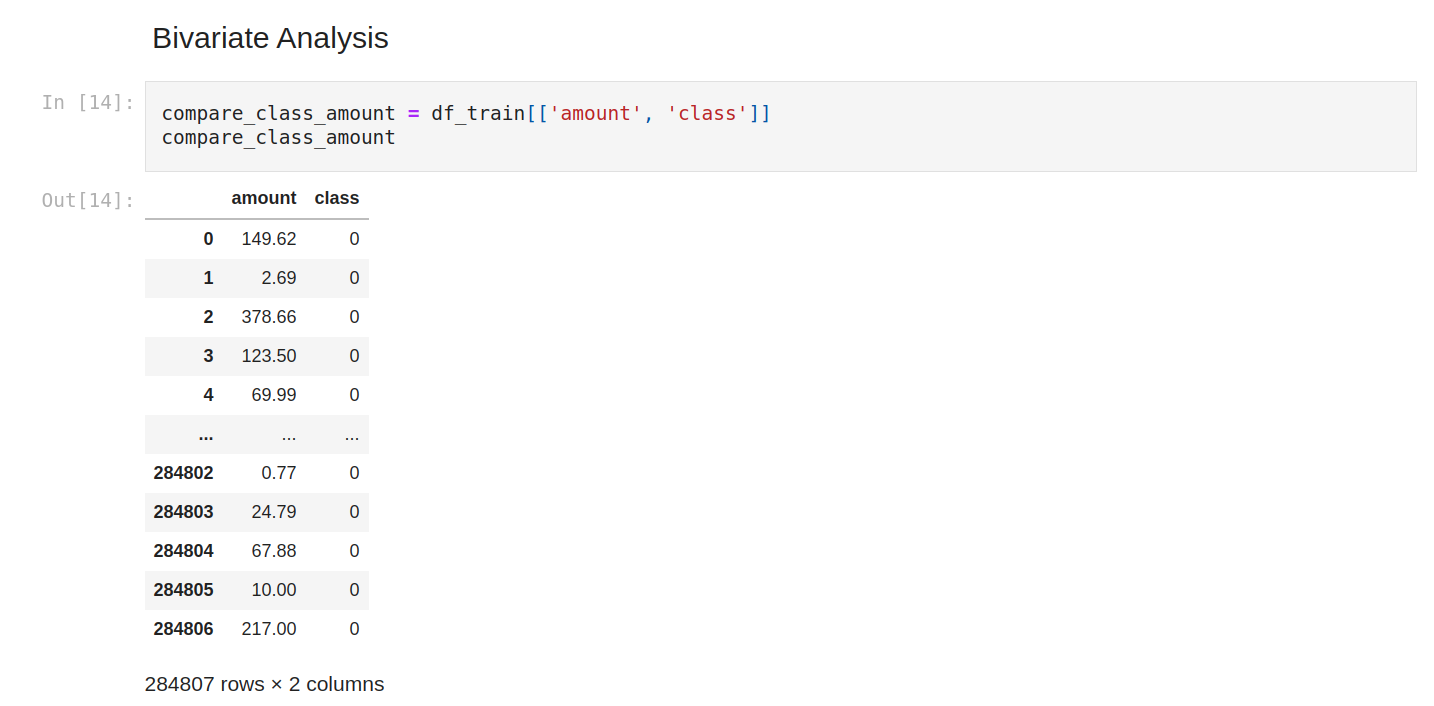And filter to show only positive class. We can see that the amount is variable.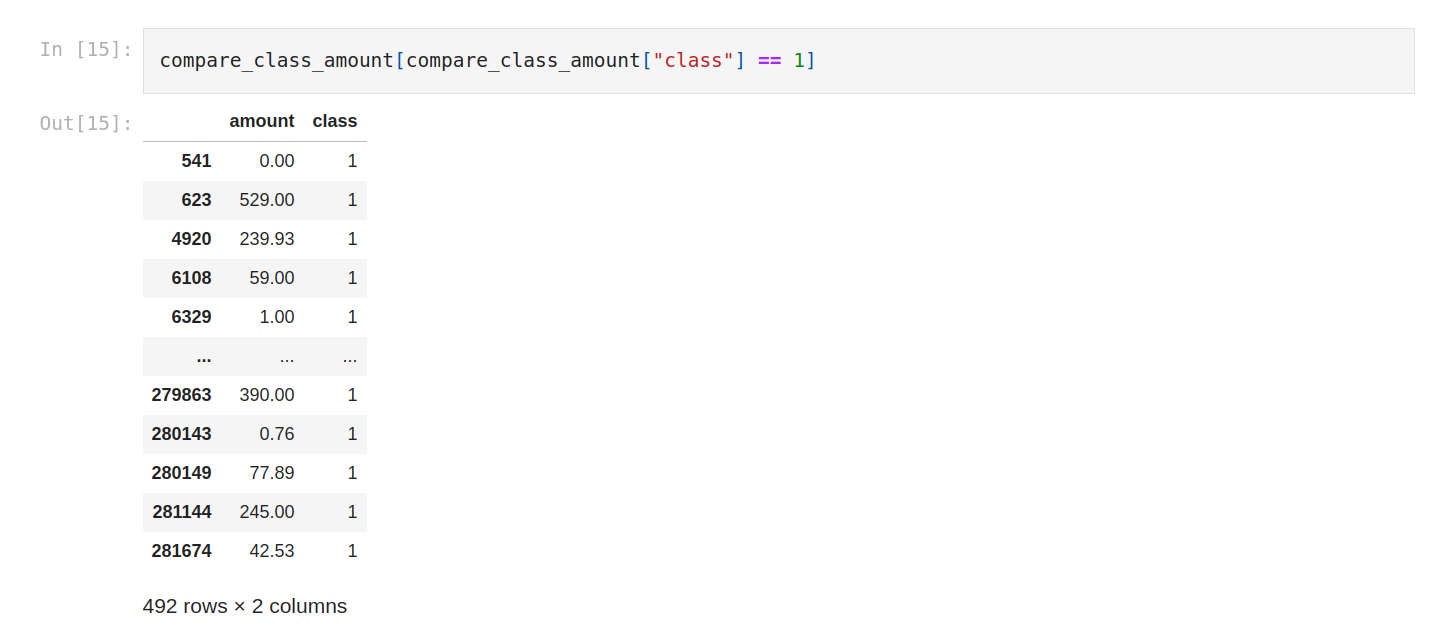Let’s check the mean.

On average the fraud is 88.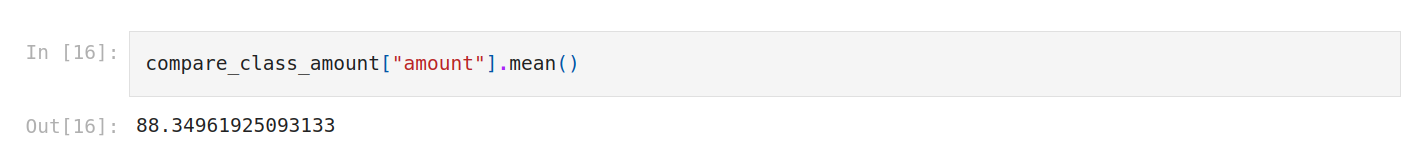Let’s check the distribution with histograms.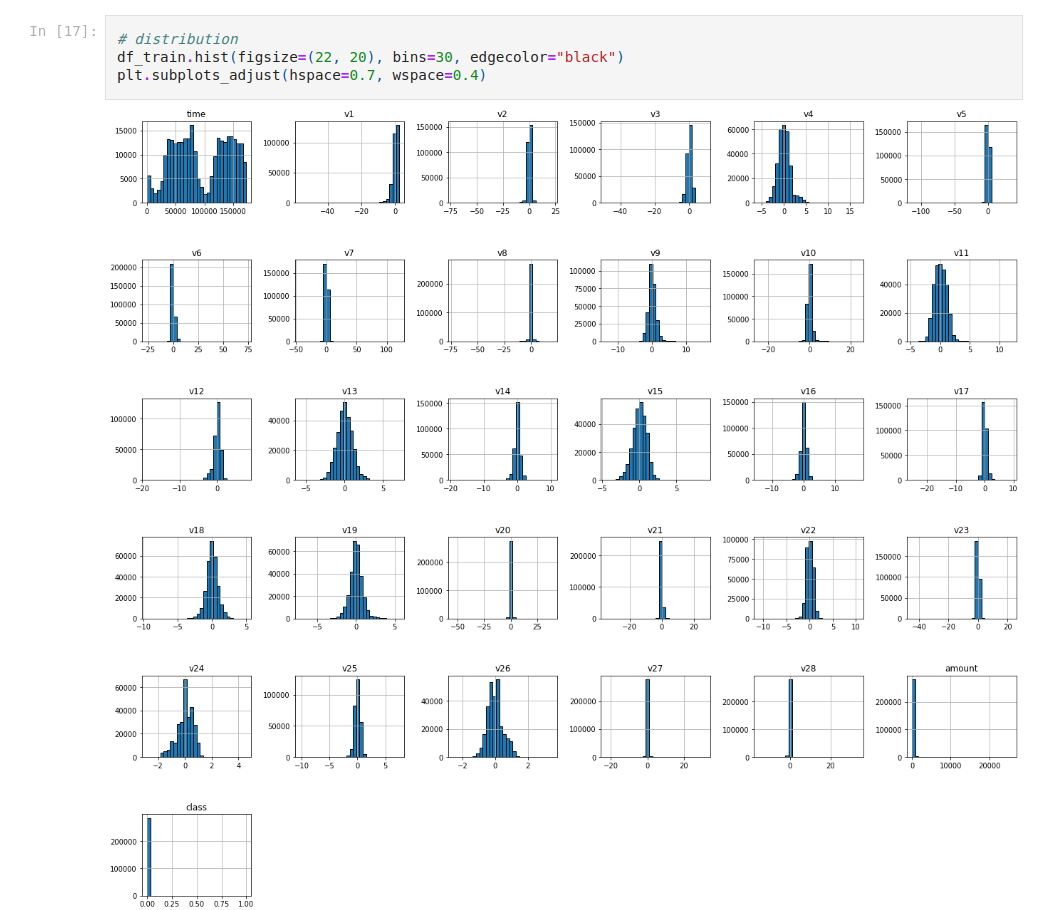One thing we can do is to check correlations.As usual; it’s better to plot it. We do that with a correlation matrix.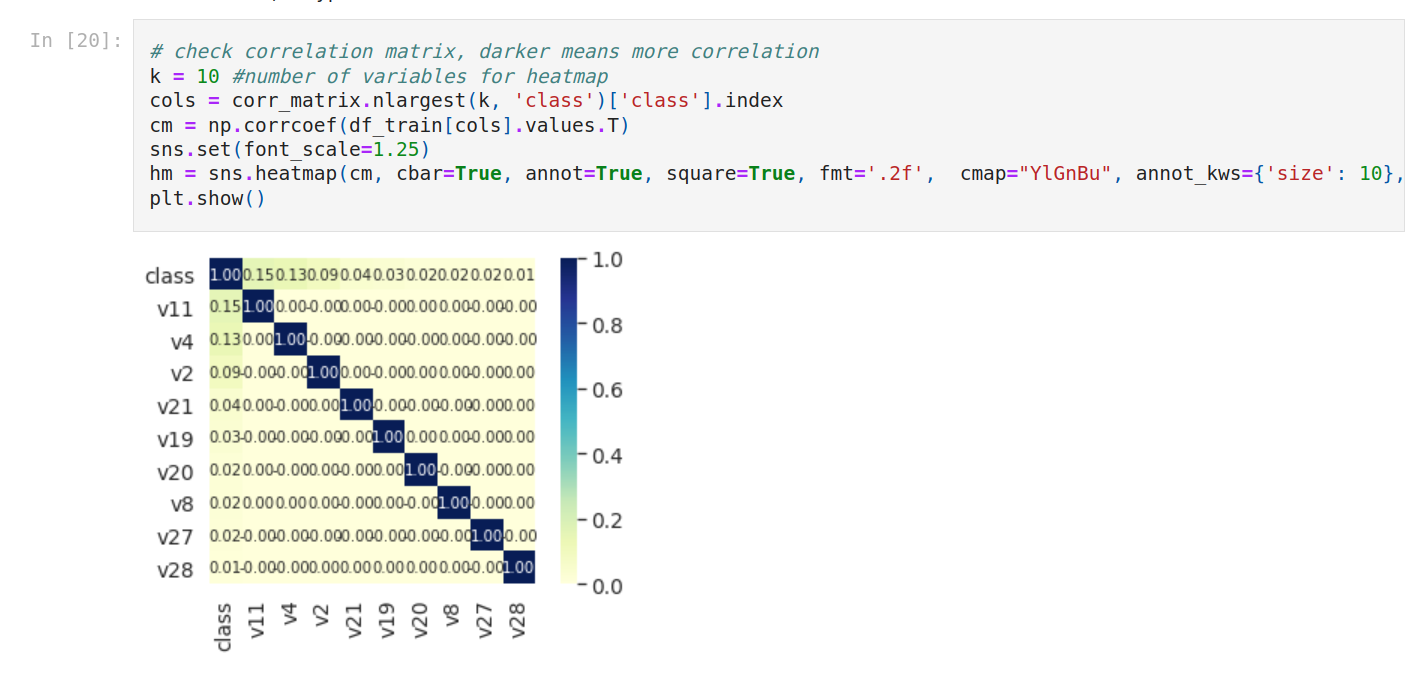Now we’re gonna check outliers. We isolate numerical columns just to show you how to do that.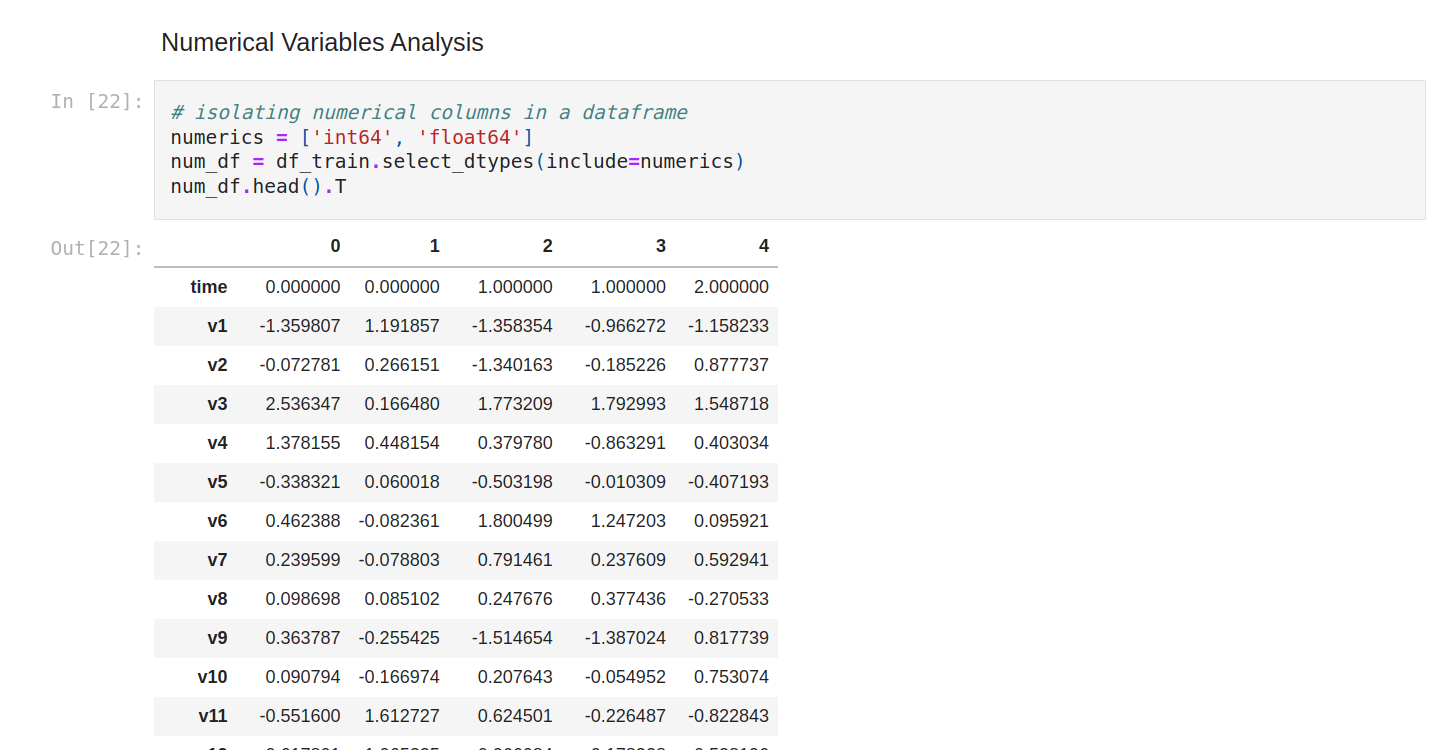Let’s do again the summary statistics of all the columns.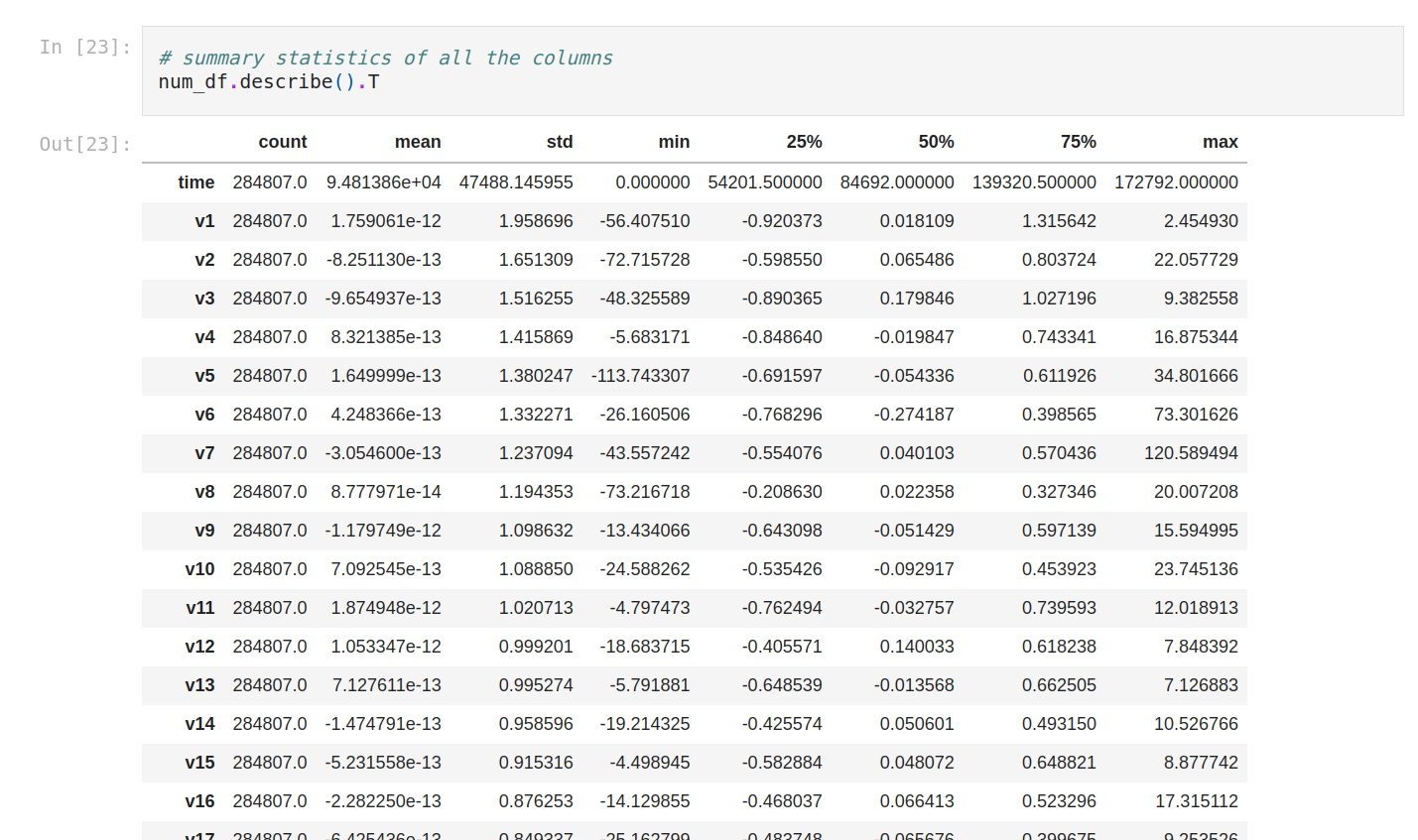We can plot the outliers with boxplots. So we do that for amount and V25.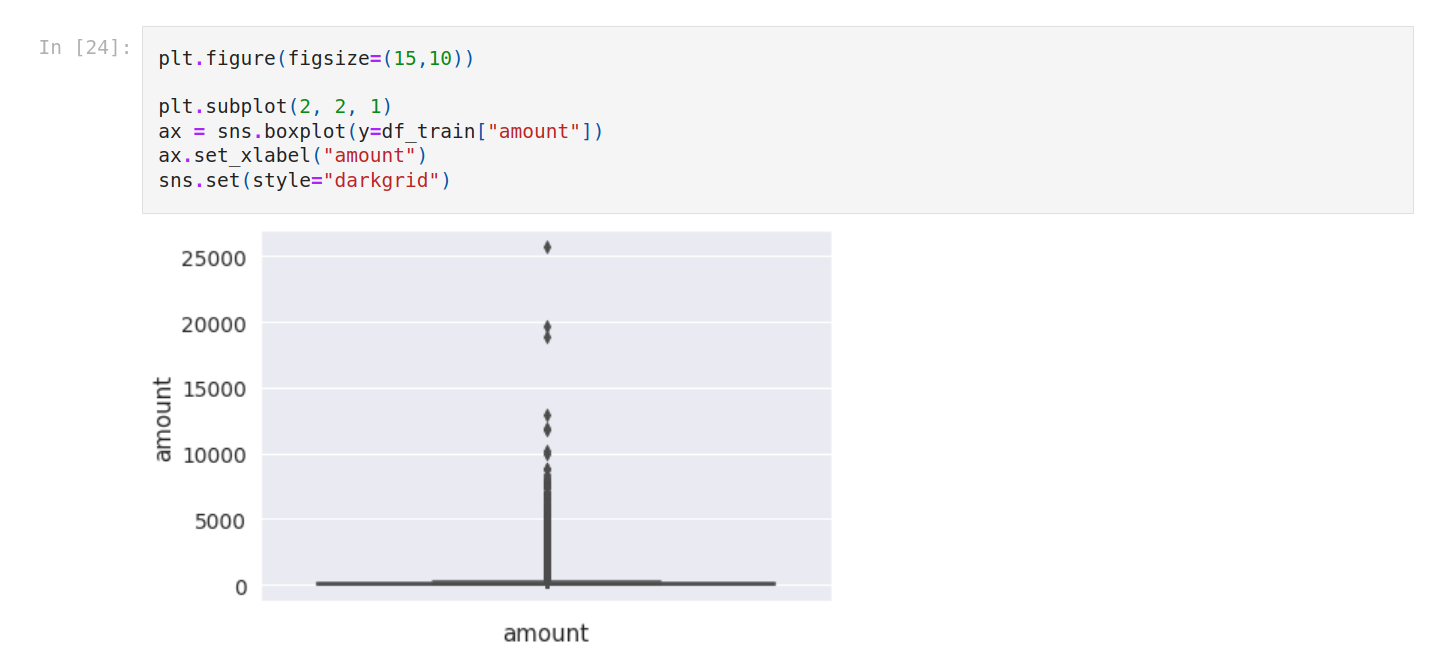Also, we calculate outlier space for both.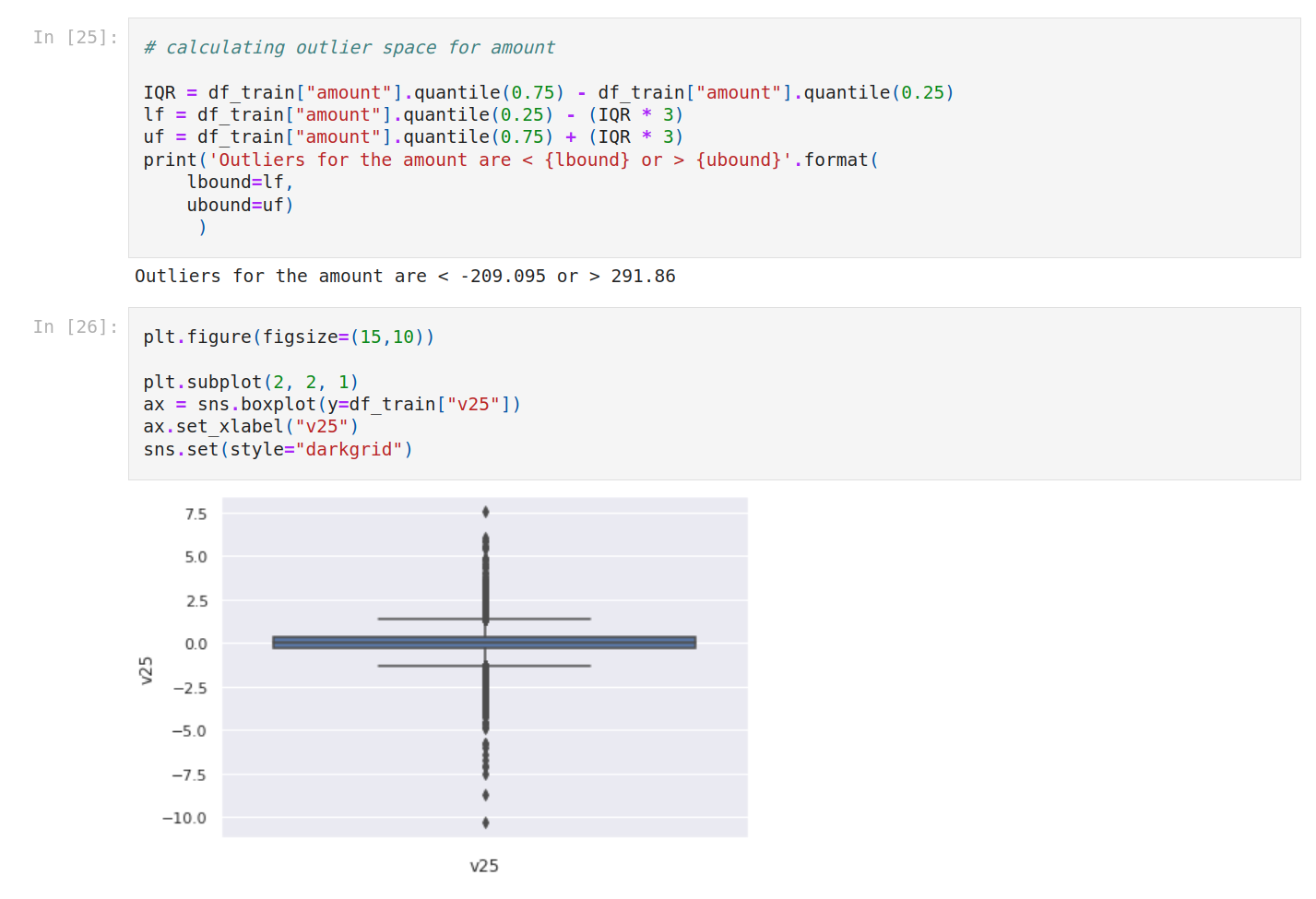### 4. Baseline model

It’s time to create our baseline model. The goal is to have a quick idea about what we can get with just a few variables.

We select 5 variables and of course without the target.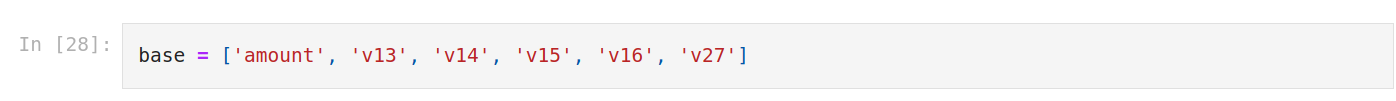So we create a function that we name baseline_model. The input is the dataset.

First we isolate the target from the target as usual (class). We get the features and we return the features and the target separately.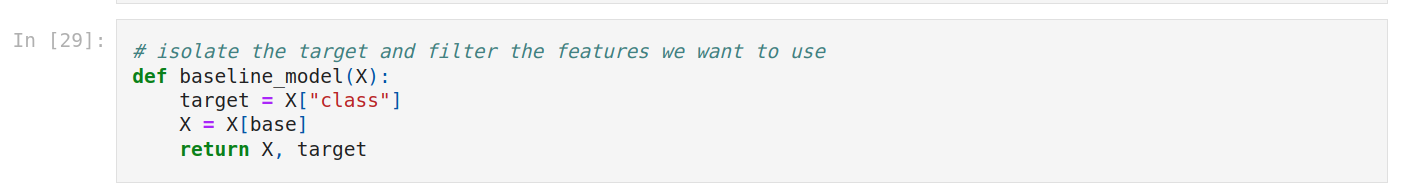We create X and y.

Let’s check X.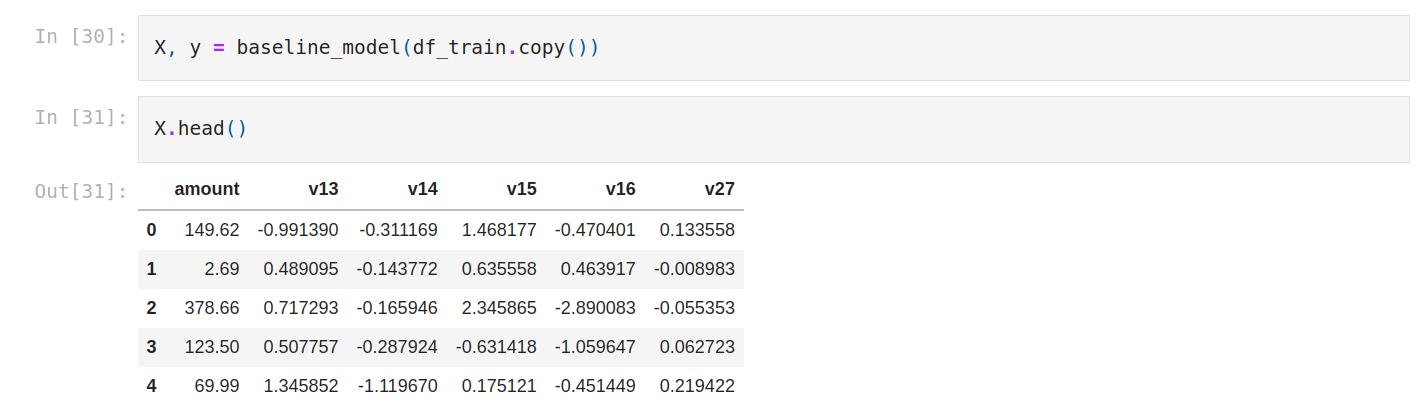Then, we import the logistic regression algorithm.

We instantiate the model, and we train it.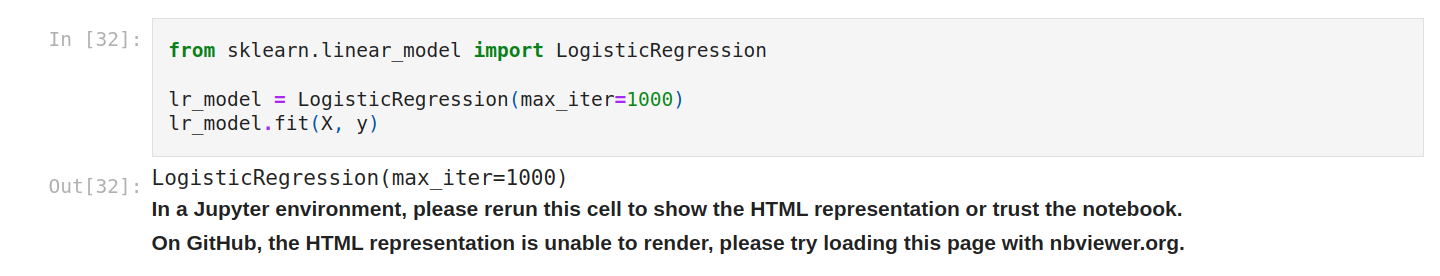We make predictions.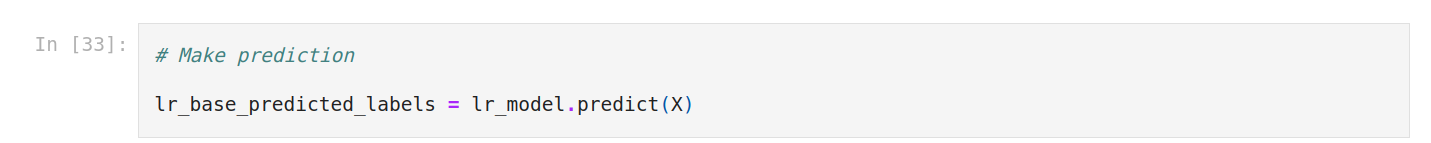We’re gonna use the confusion matrix. A confusion matrix is a table that is used to define the performance of a classification algorithm.

Precision-Recall is a useful measure of the success of prediction when the classes are very imbalanced. In information retrieval, precision is a measure of result relevancy, while recall is a measure of how many truly relevant results are returned.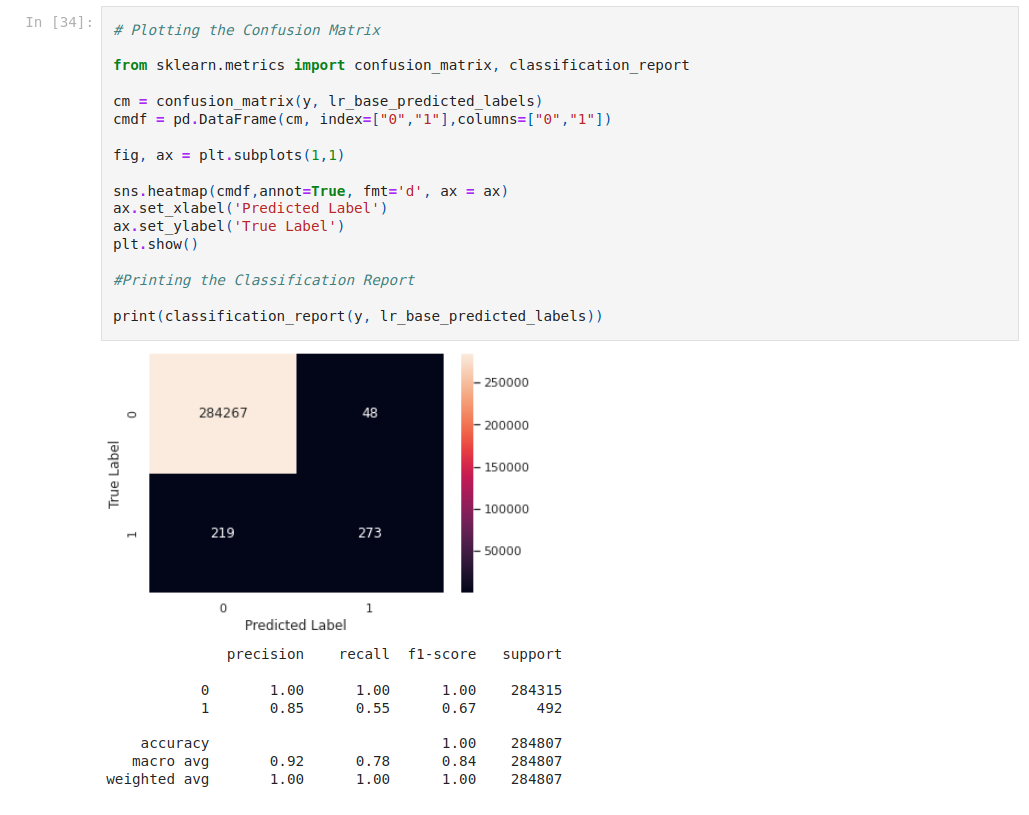### 5. Feature engineering

It’s time to do feature engineering. We start with feature scaling. let's use describe.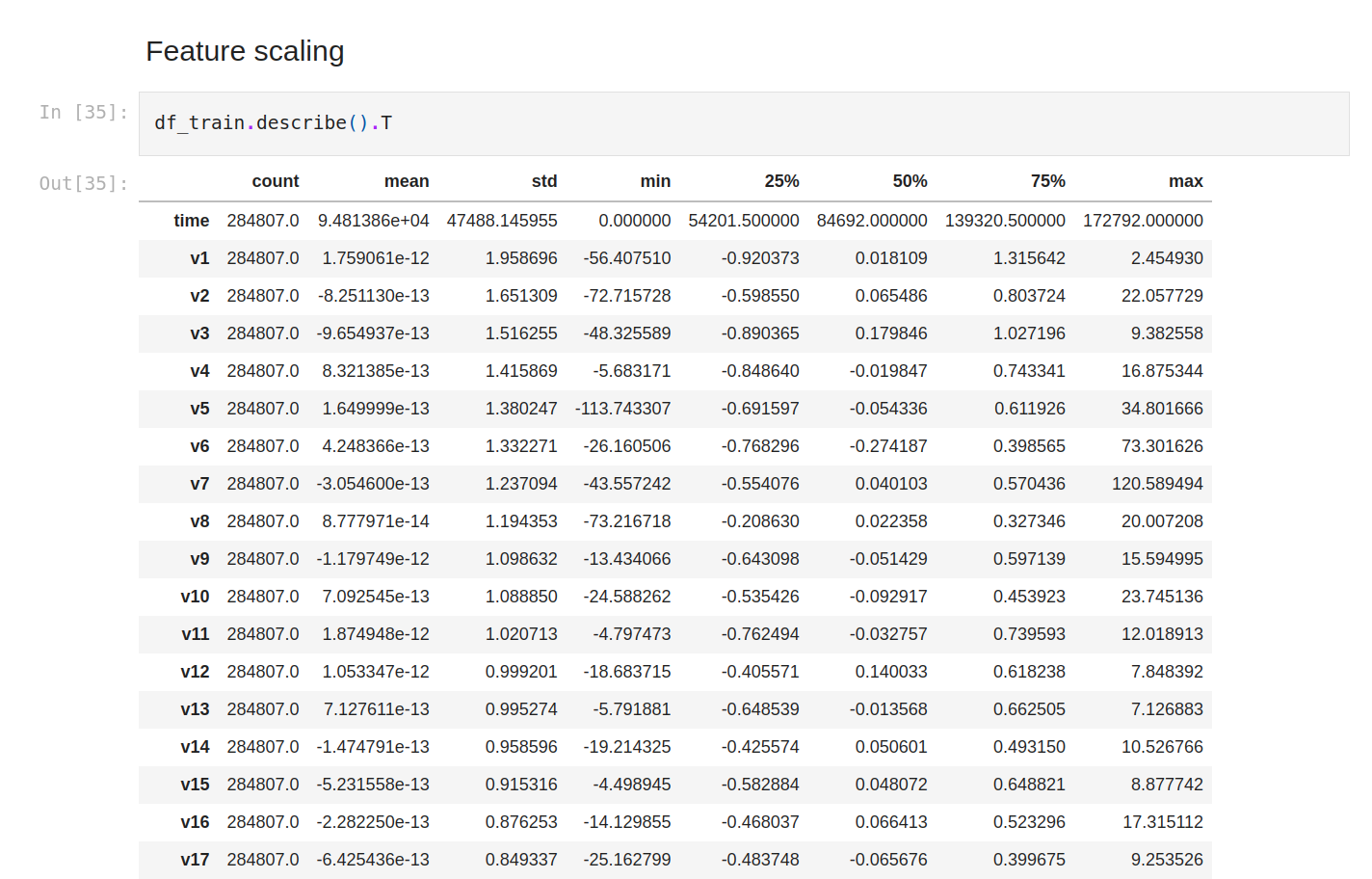Mean, std, min and max are very different from each other, so let's standardize. We’re gonna use StandardScaler and MinMaxScaler.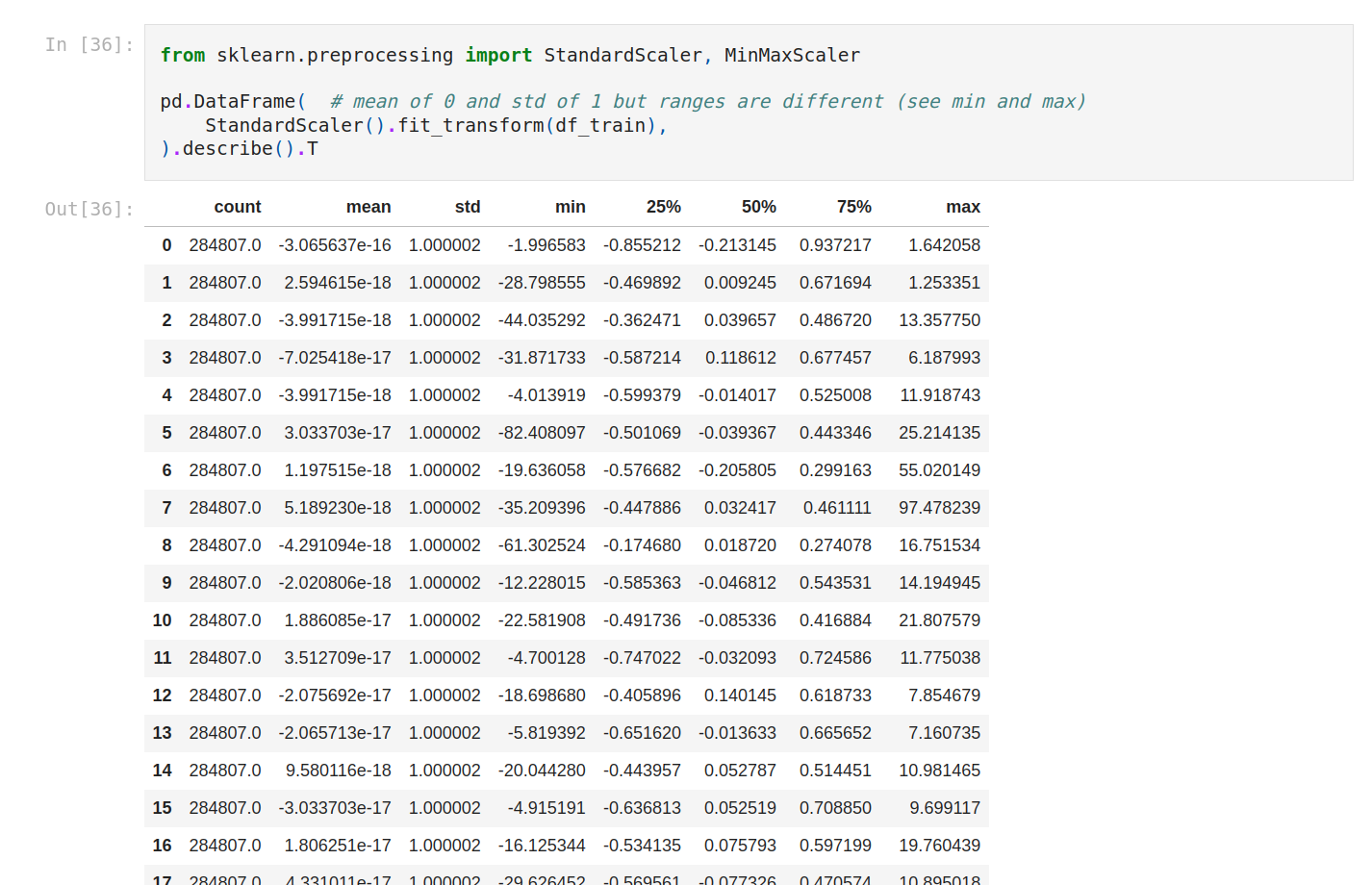With MinMaxScaler everything is on the same scale.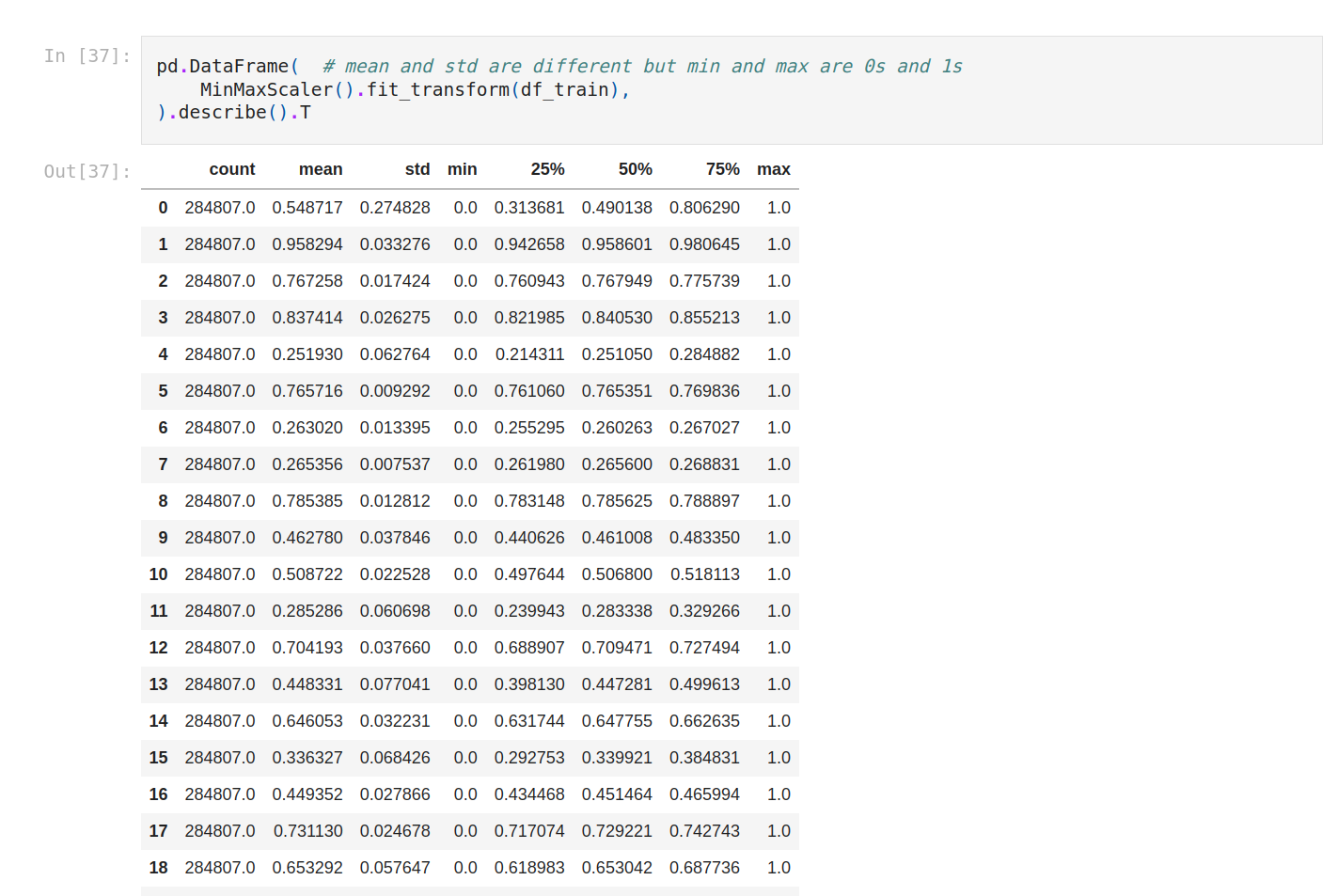Now we can update the model with the other features.

Let’s save the dataset. Now we get the new one.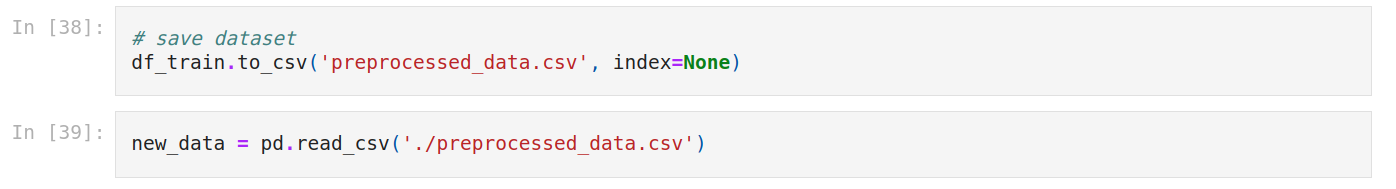So we create a function named update_model. Pretty much the same as the first one but this time we drop class.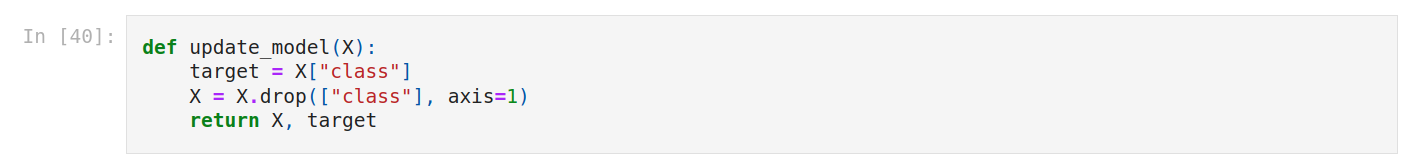We update X and y.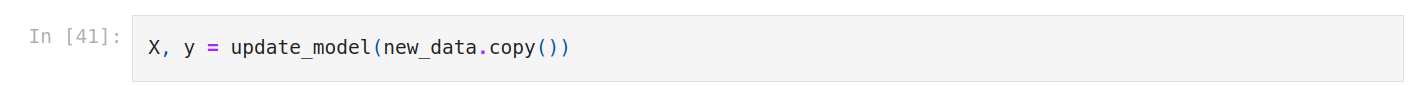Then we fit the model.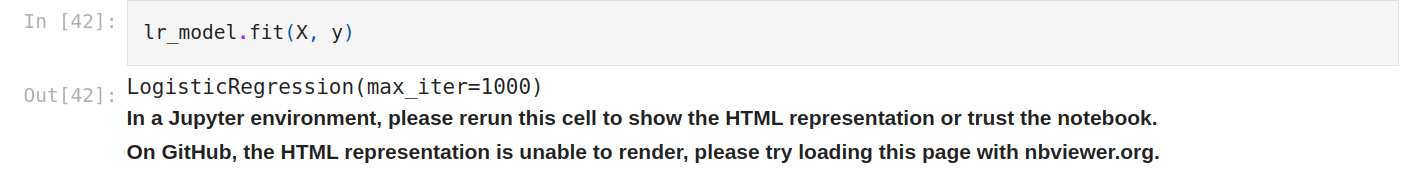We make predictions.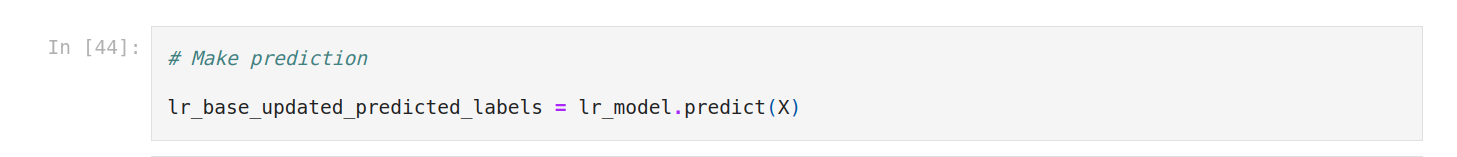We plot again the confusion matrix. It seems worse.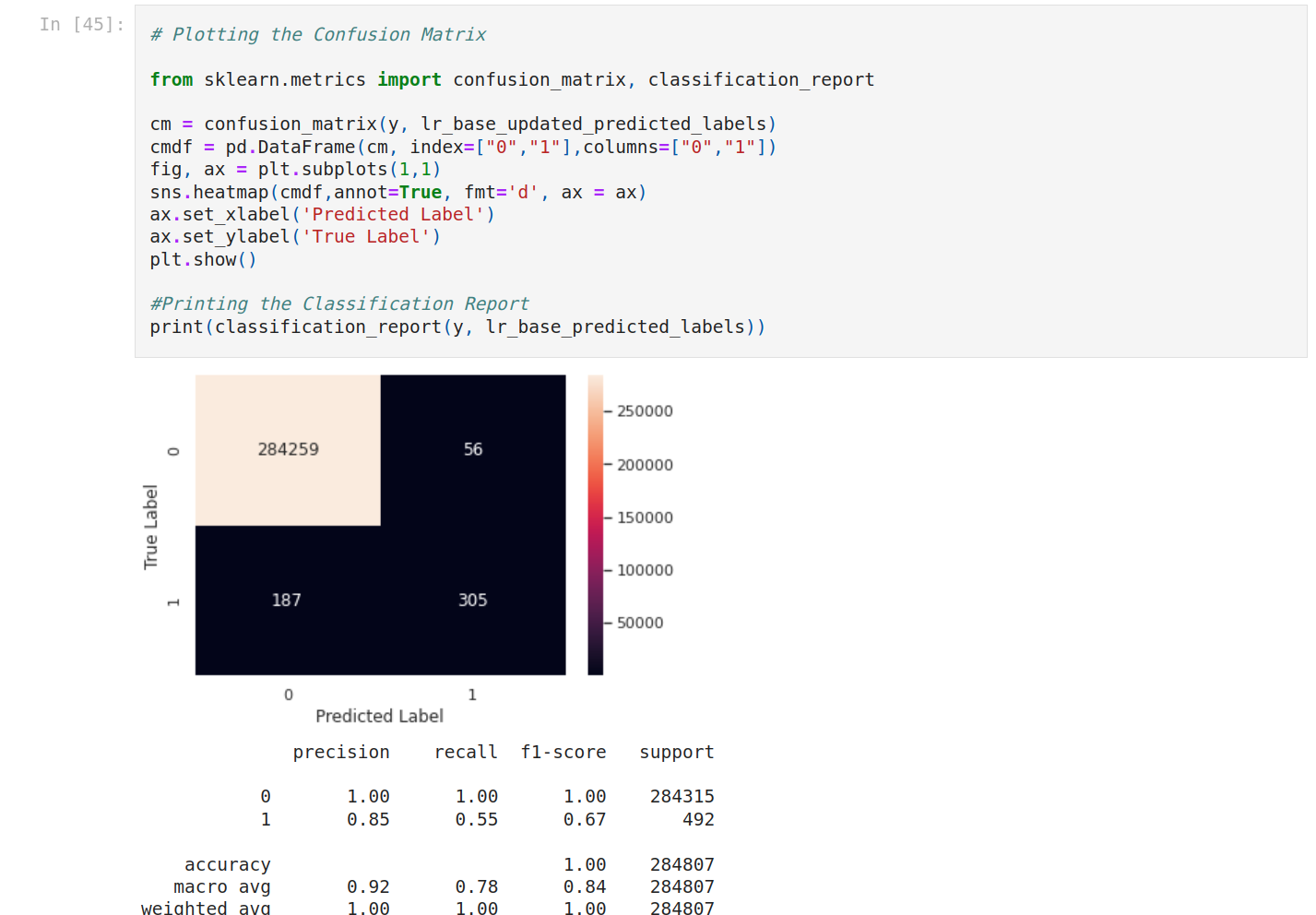Let’s see the coefficients (weight of variables).

We’re gonna use feature_names_in_.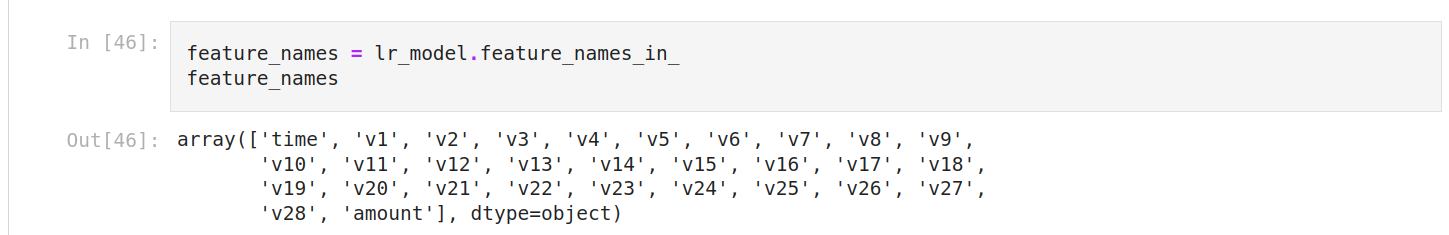We can display an array and sort it.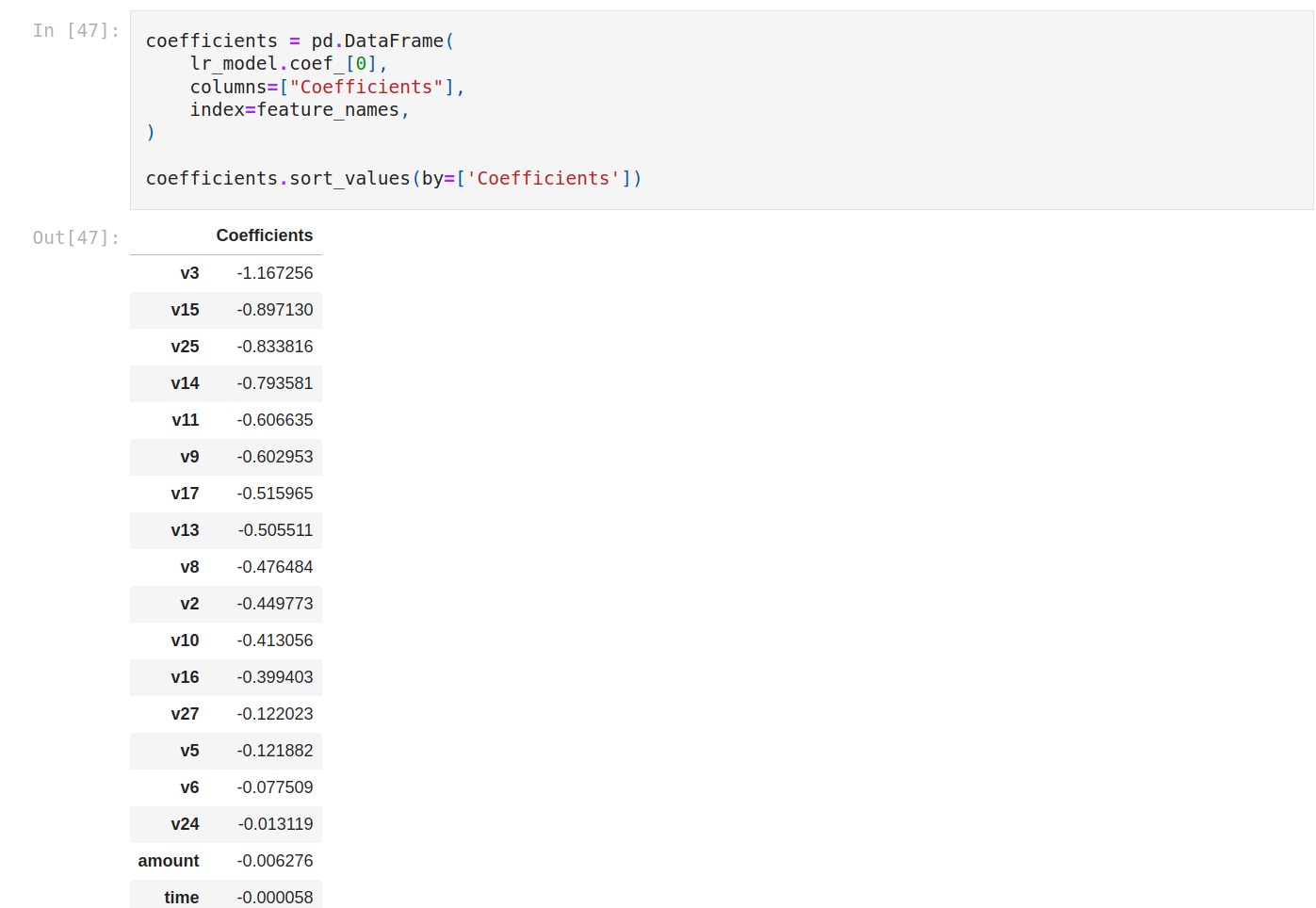Let’s plot it to see better.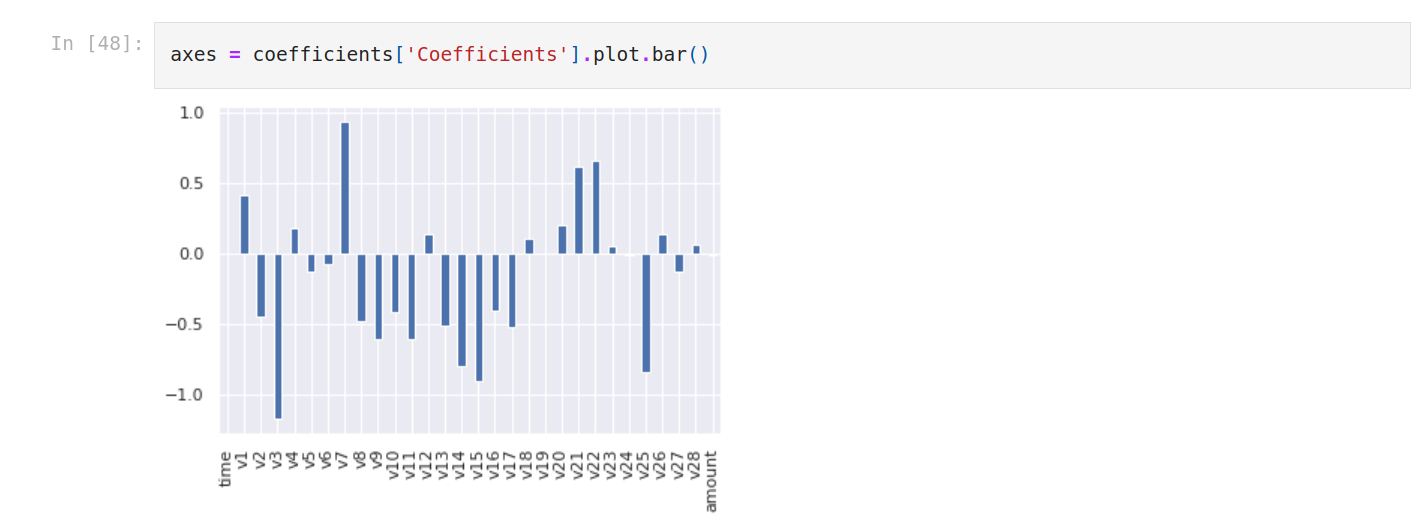There are many very discriminating features especially V3, V15, V25 are very discriminating. amount is neutral. V7, V22 and V21 have a positive impact.

When we train our model on all features, the bias term is -3.29. The reason why the sign before the bias term is negative is the class balance. Meaning the probability of non-fraudulent on average is a little high.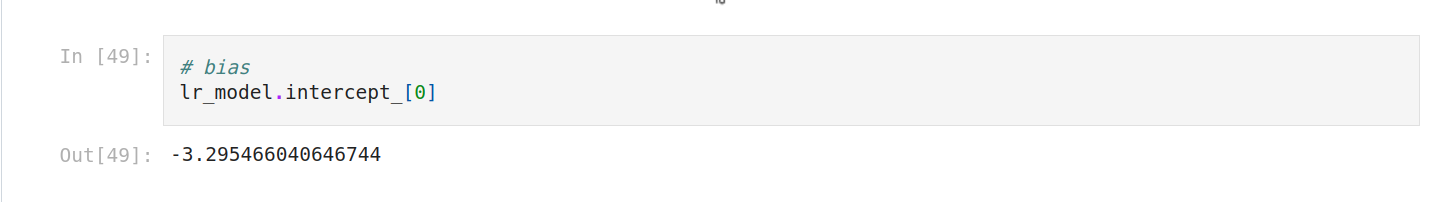### 6. Training other models

We gonna use

• Decision tree
• Naive Bayes
• Support vector machine (SVM)
• Random Forest

First, we split the dataset into 3 parts (train, validation, and test). So first train, test, then validation, test.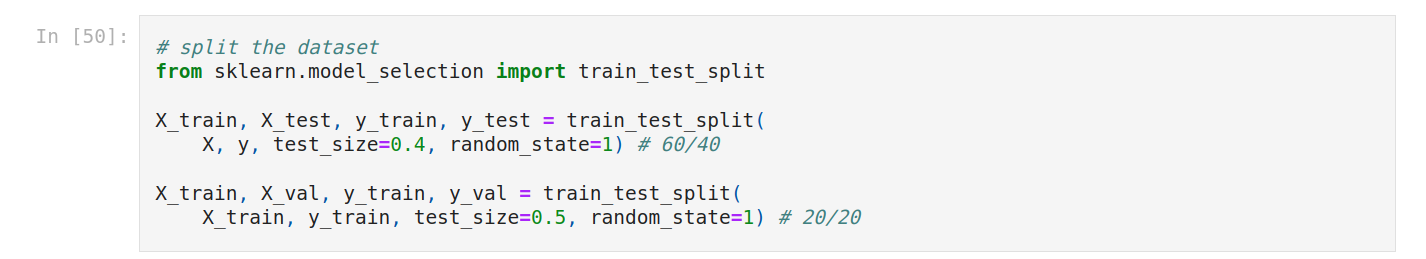Now we fit again logistic regression.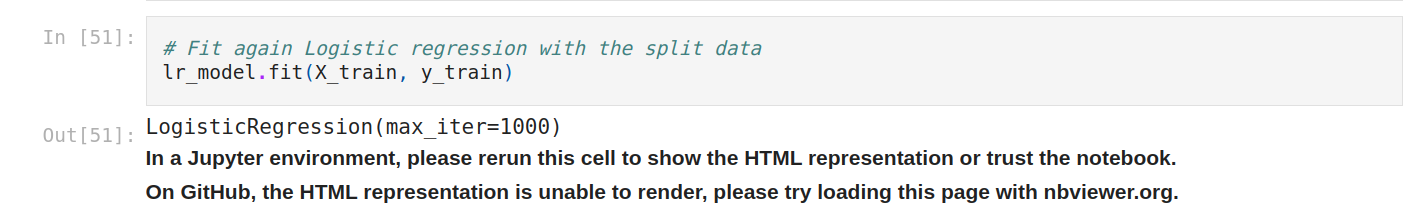And we instantiate the other algos, then train them. We evaluate them.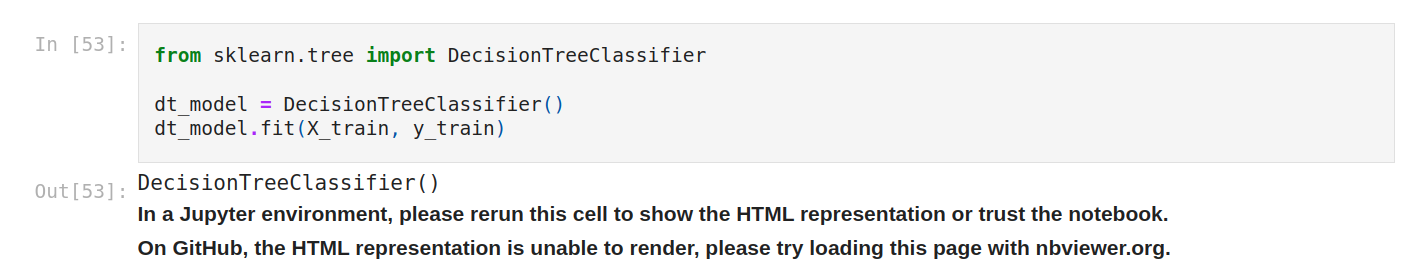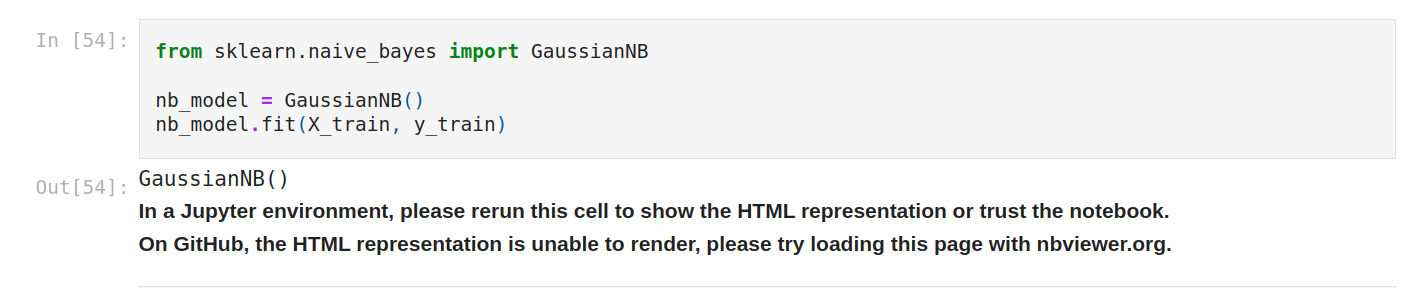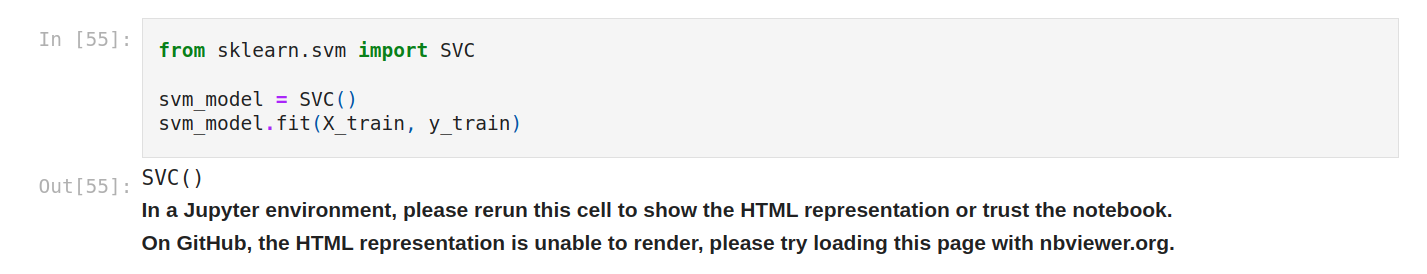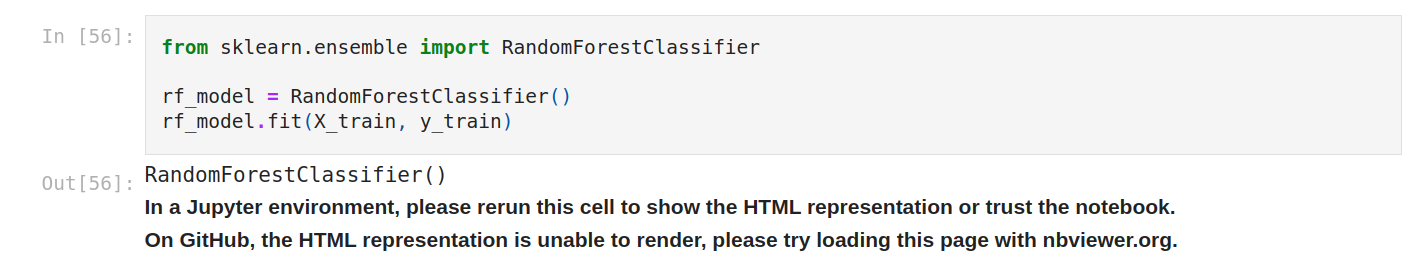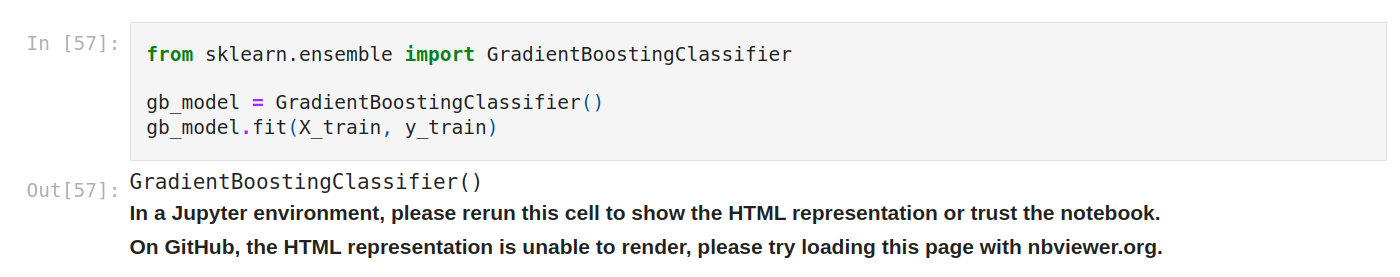### 7. Evaluating models

First, we make predictions on validation data for each model.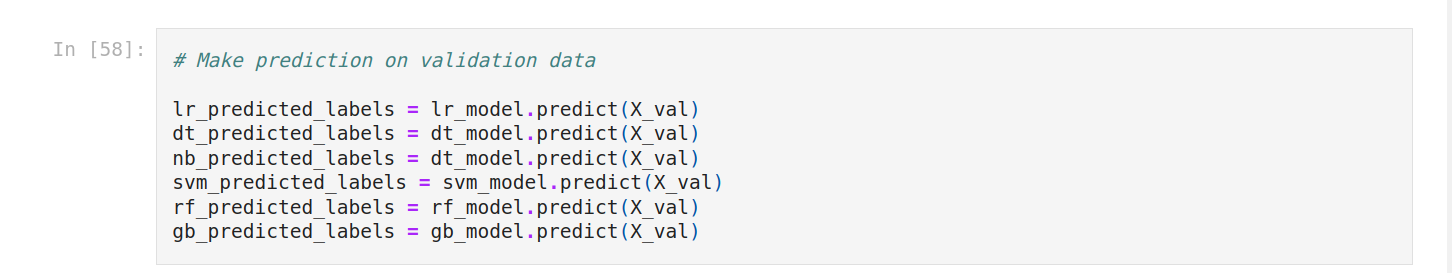Then plot the confusion matrix for each model.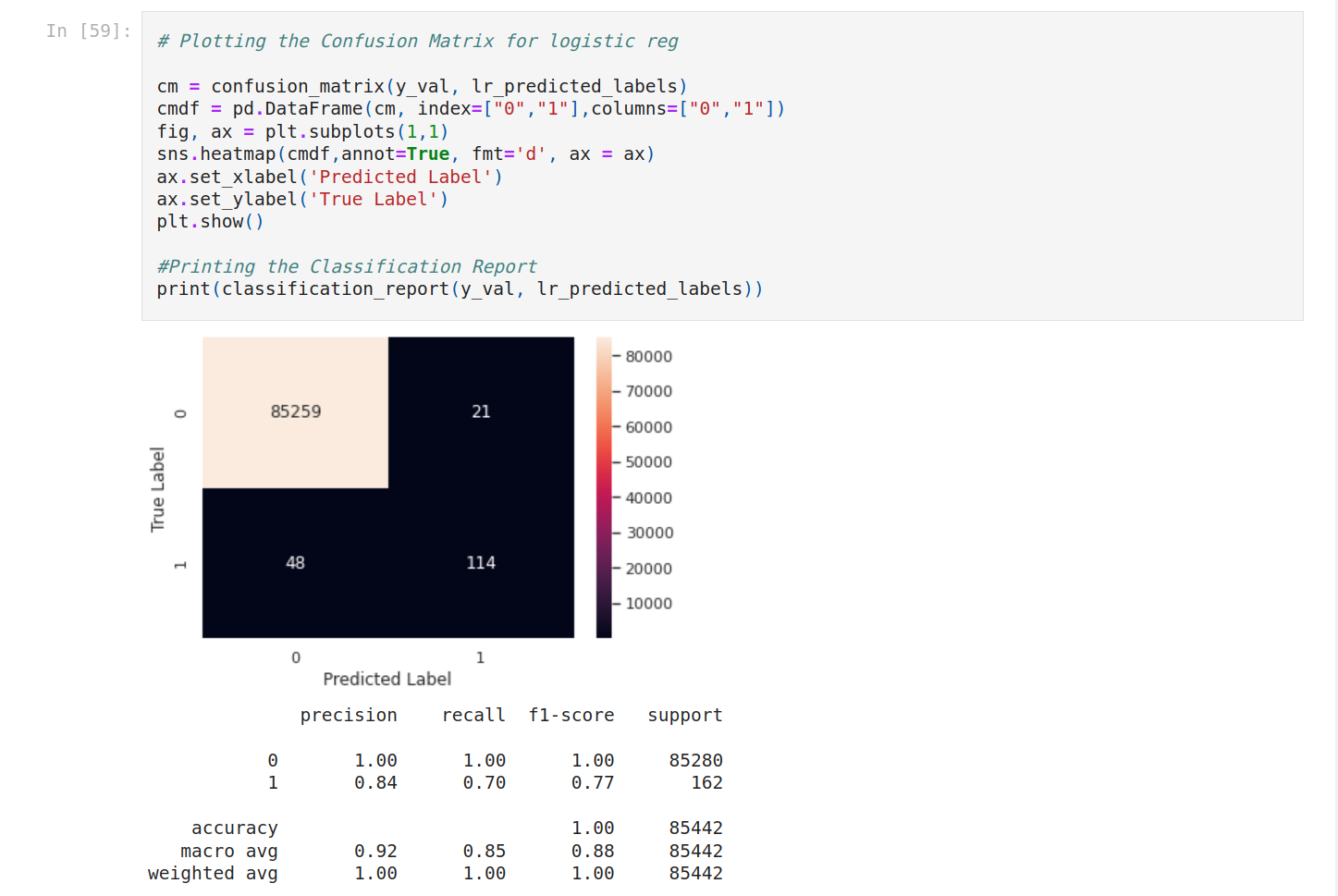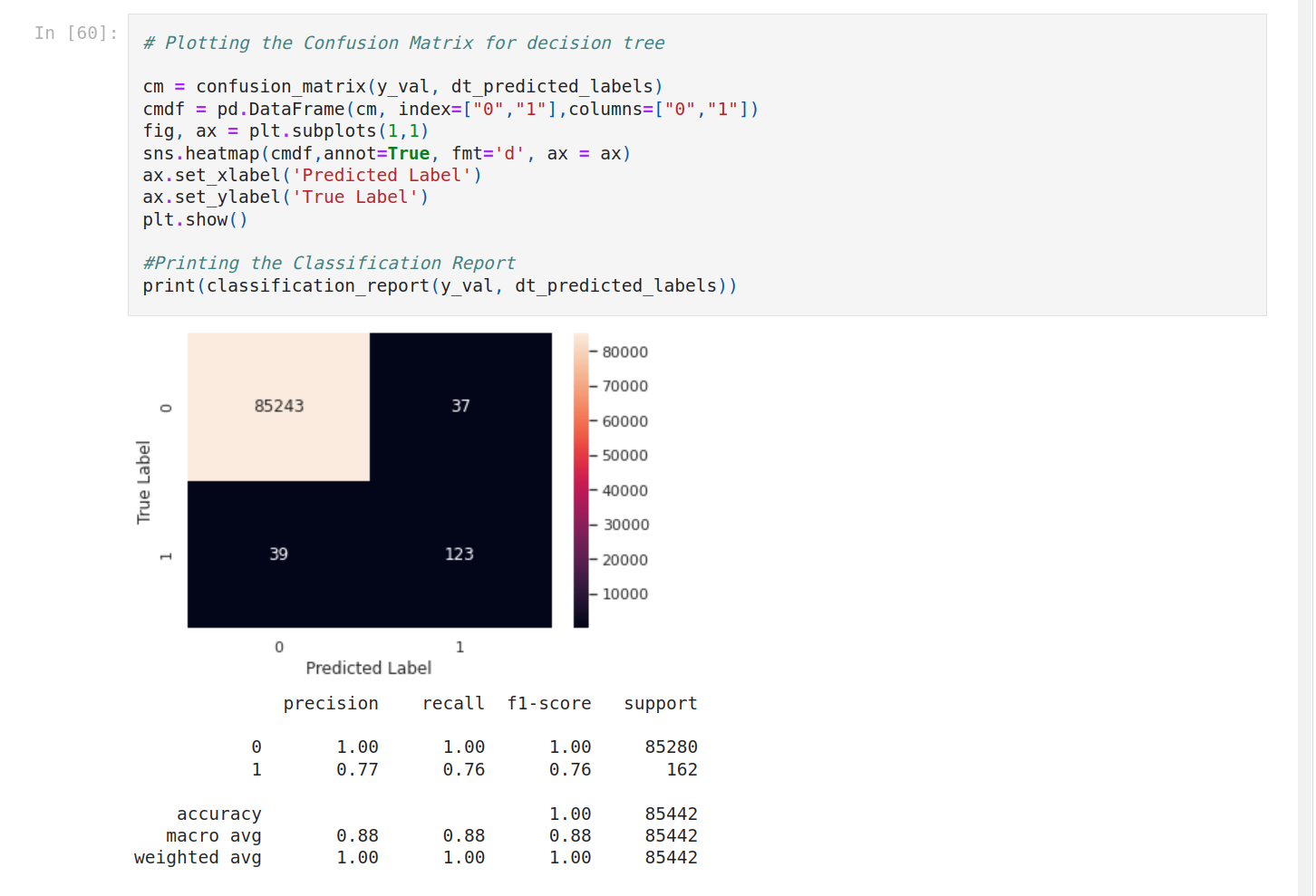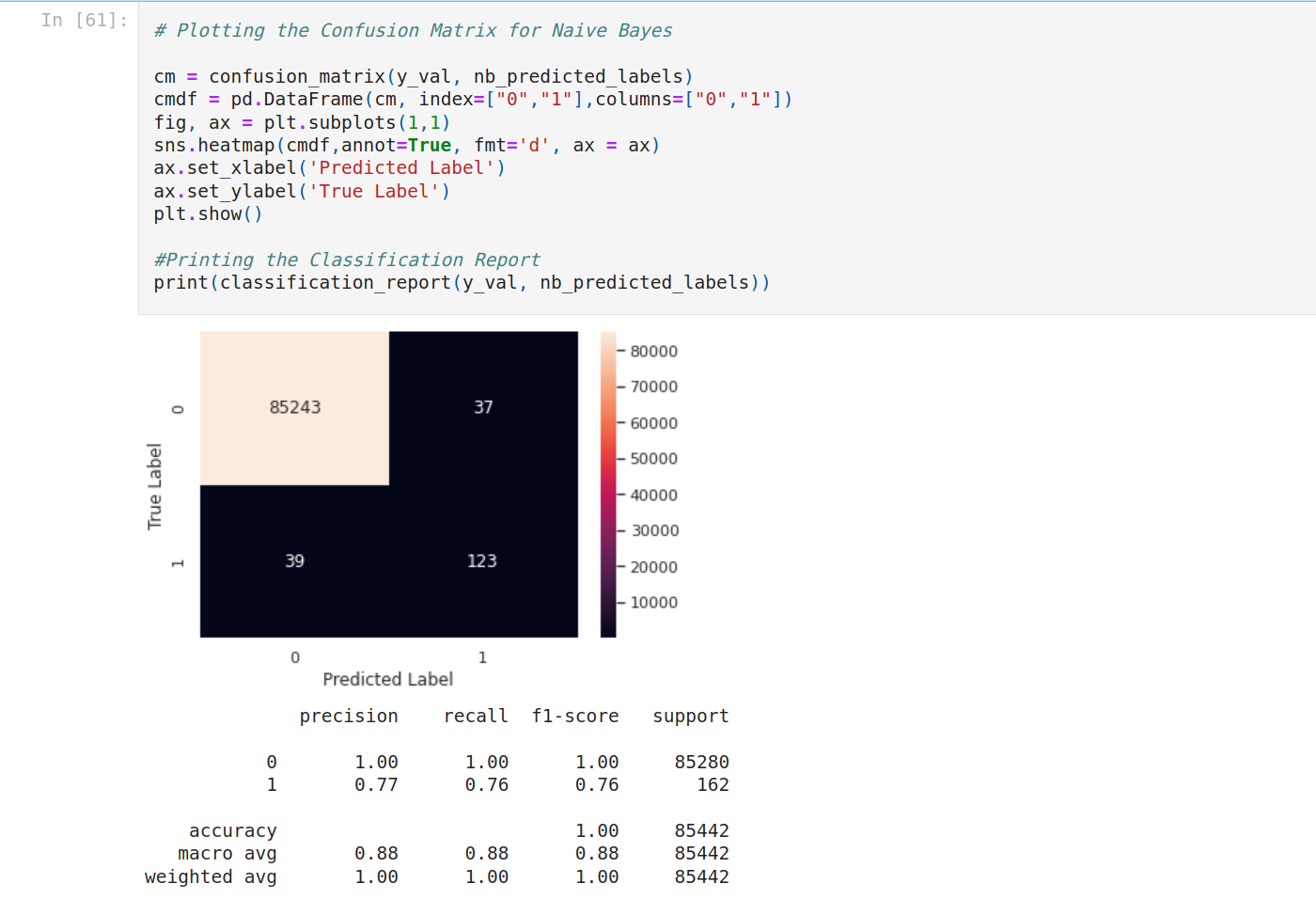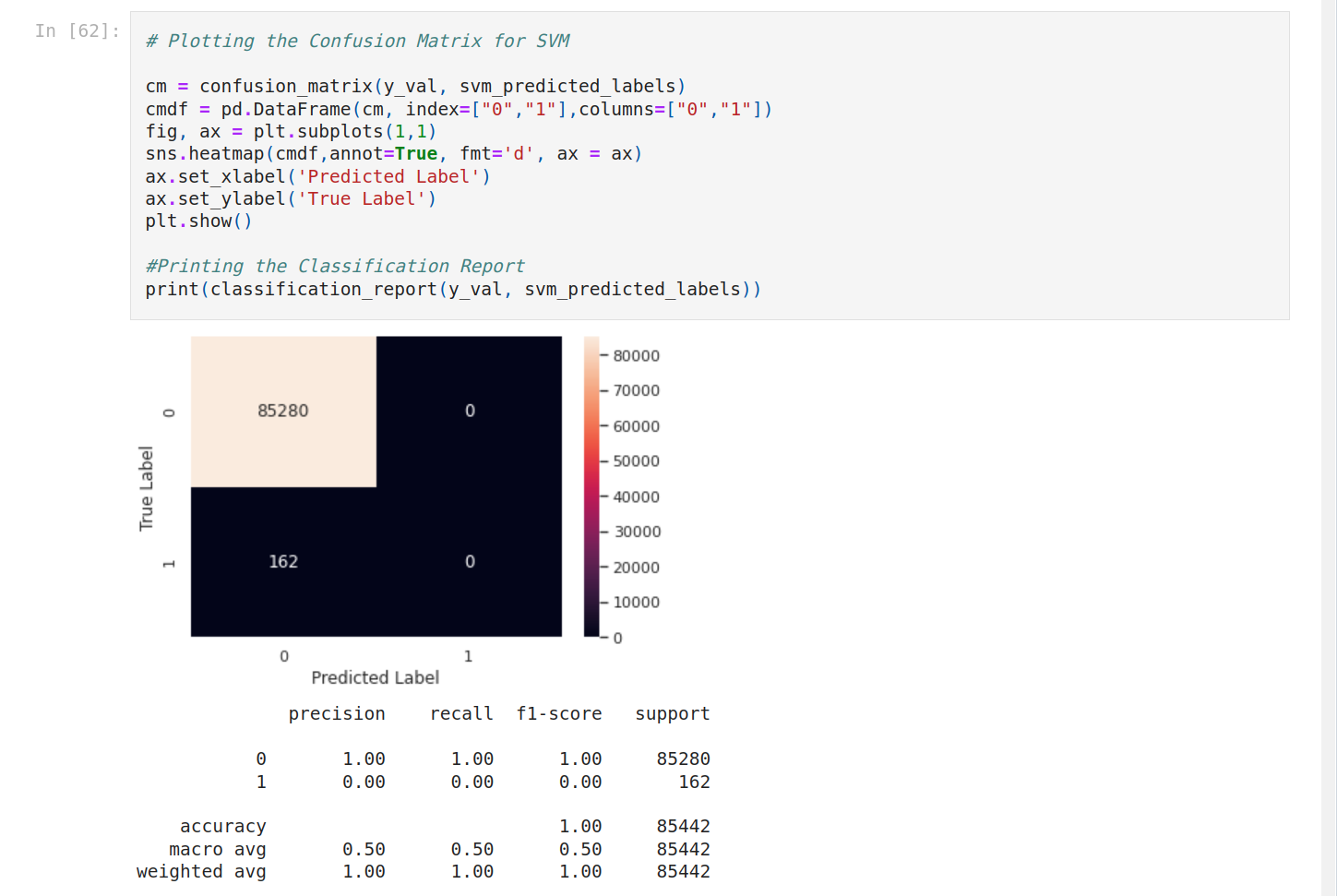It seems to have a problem with SVM. Let's focus on the other models.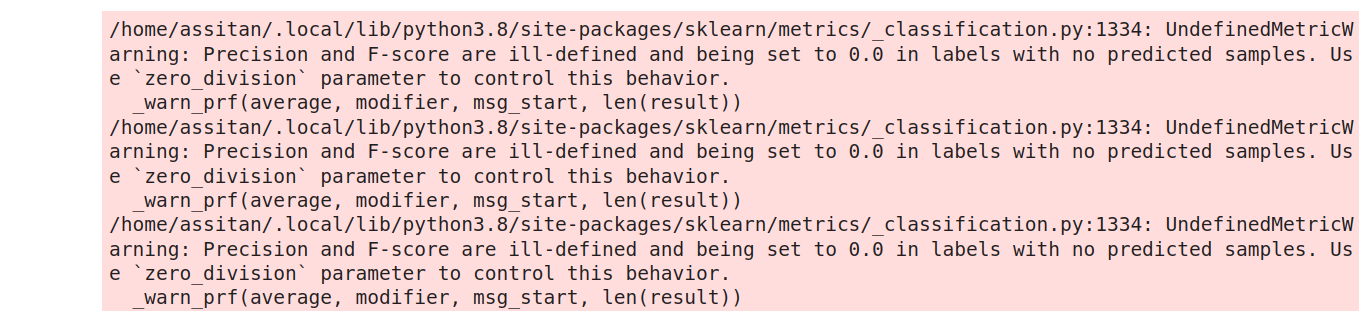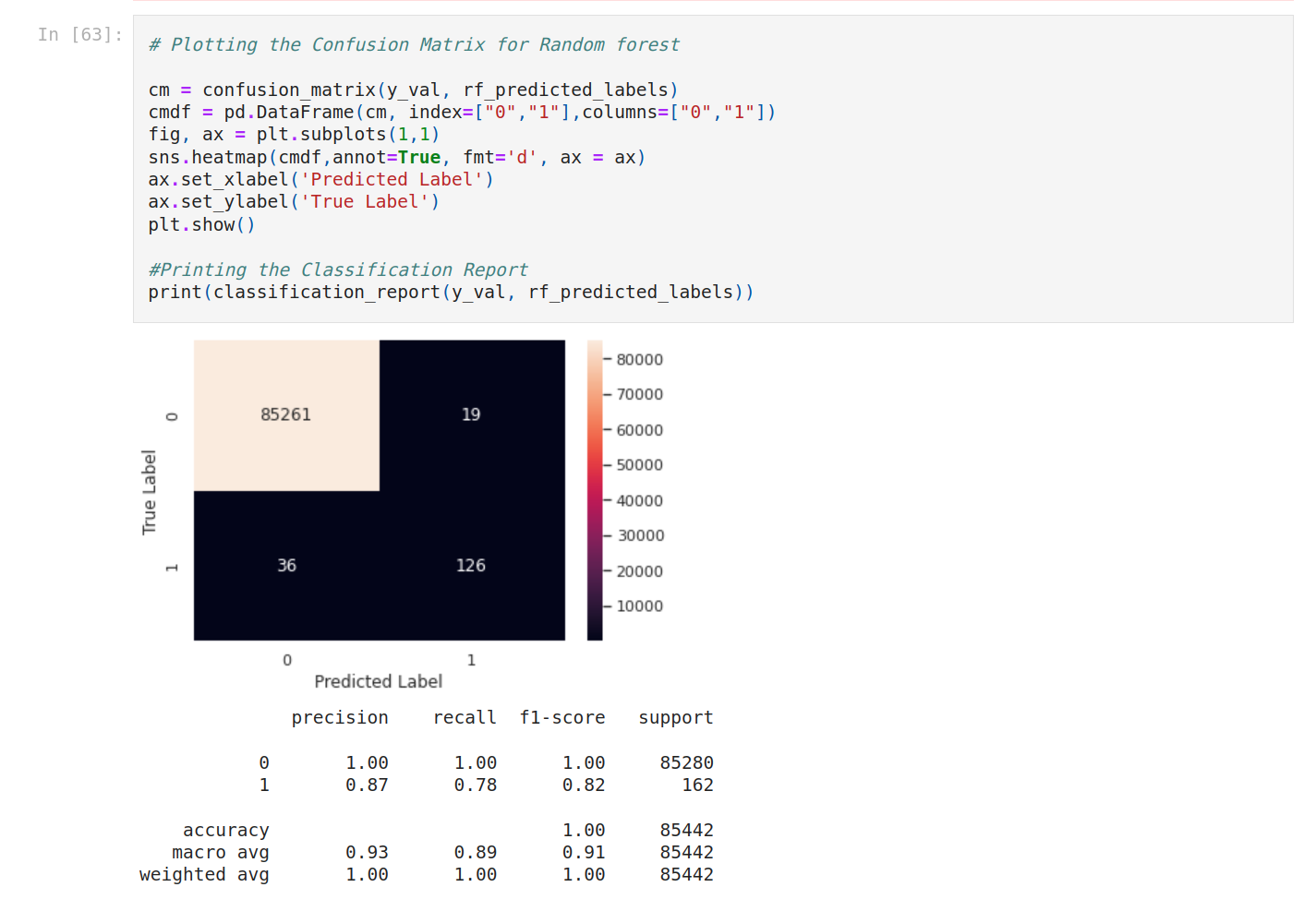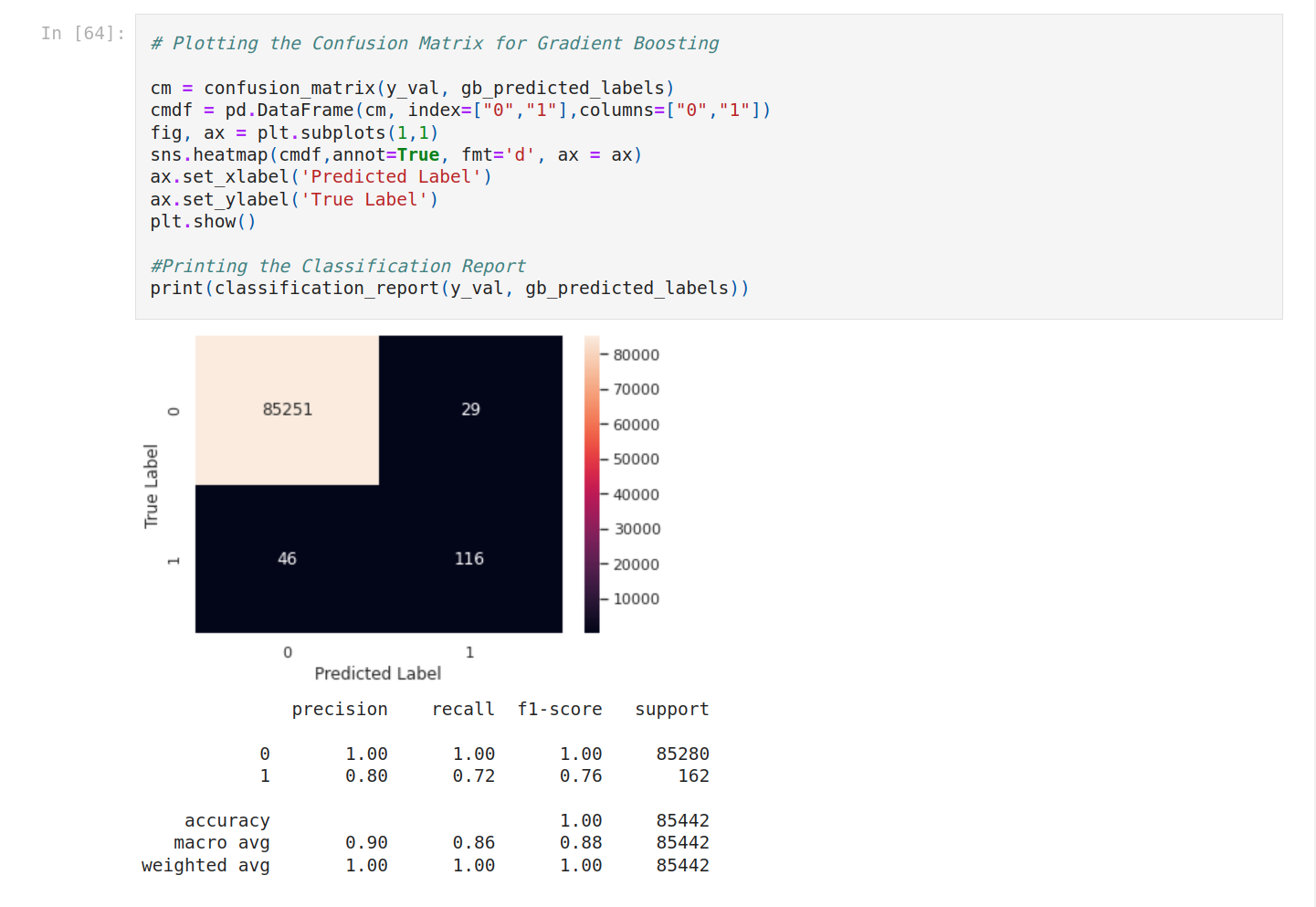To see better, let’s compare the F1-score.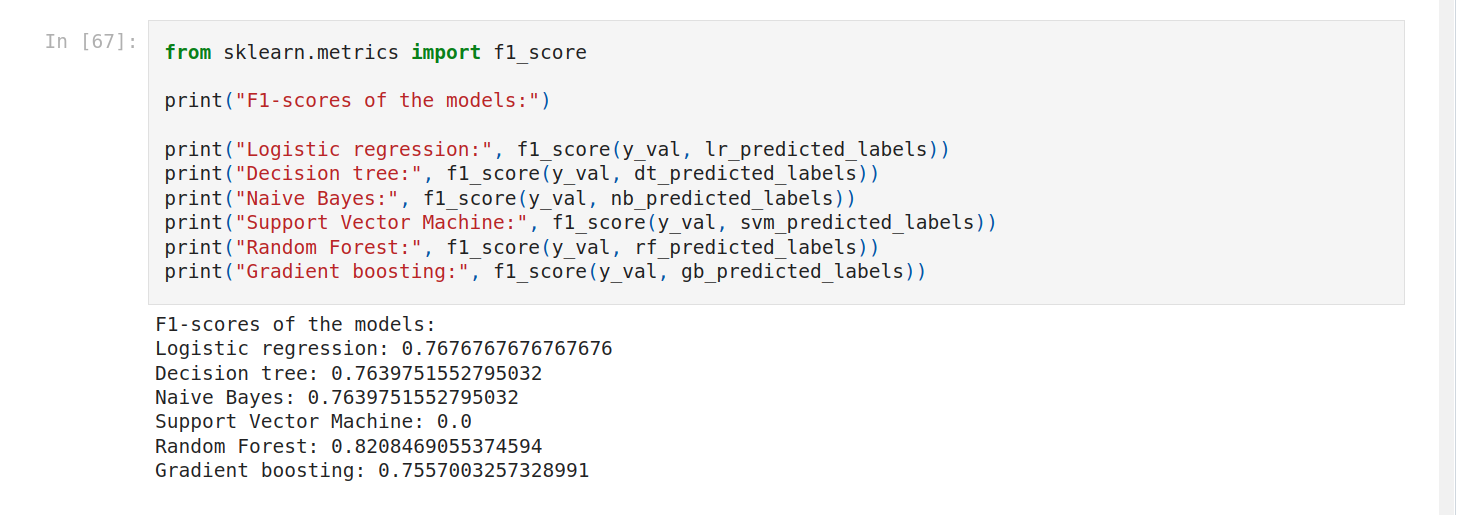Random forest is the best score!

### Hyperparameter tuning

We can do hyperparameter tuning to improve the score. I want to improve the score of logistic regression and random forest. We can use random RandomizedSearchCV (for logistic regression) and GridSearchCV. First we create a grid.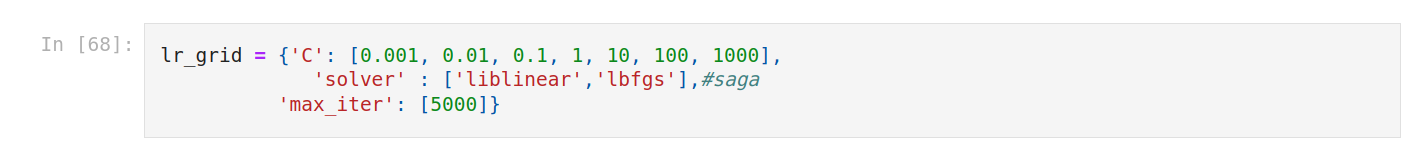Then set up random hyperparameter search for Logistic regression. Train the new model.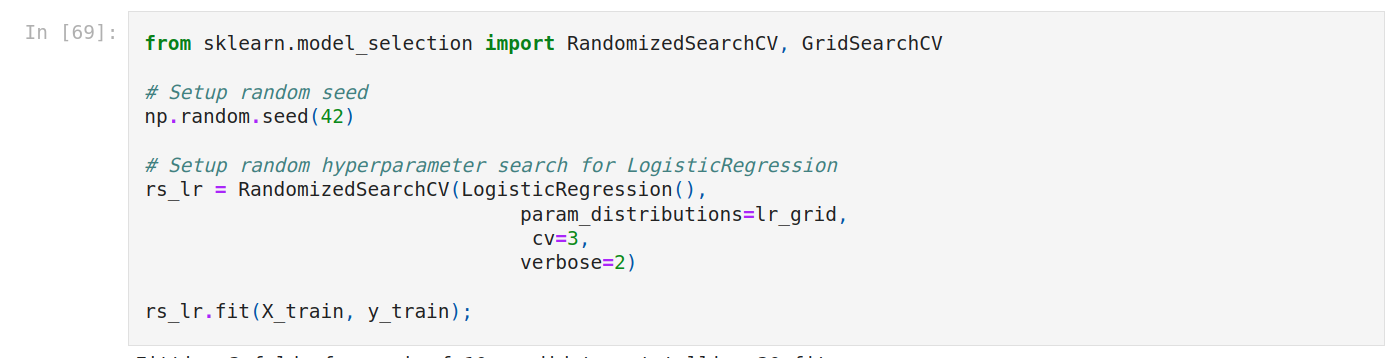We have to wait a few minutes.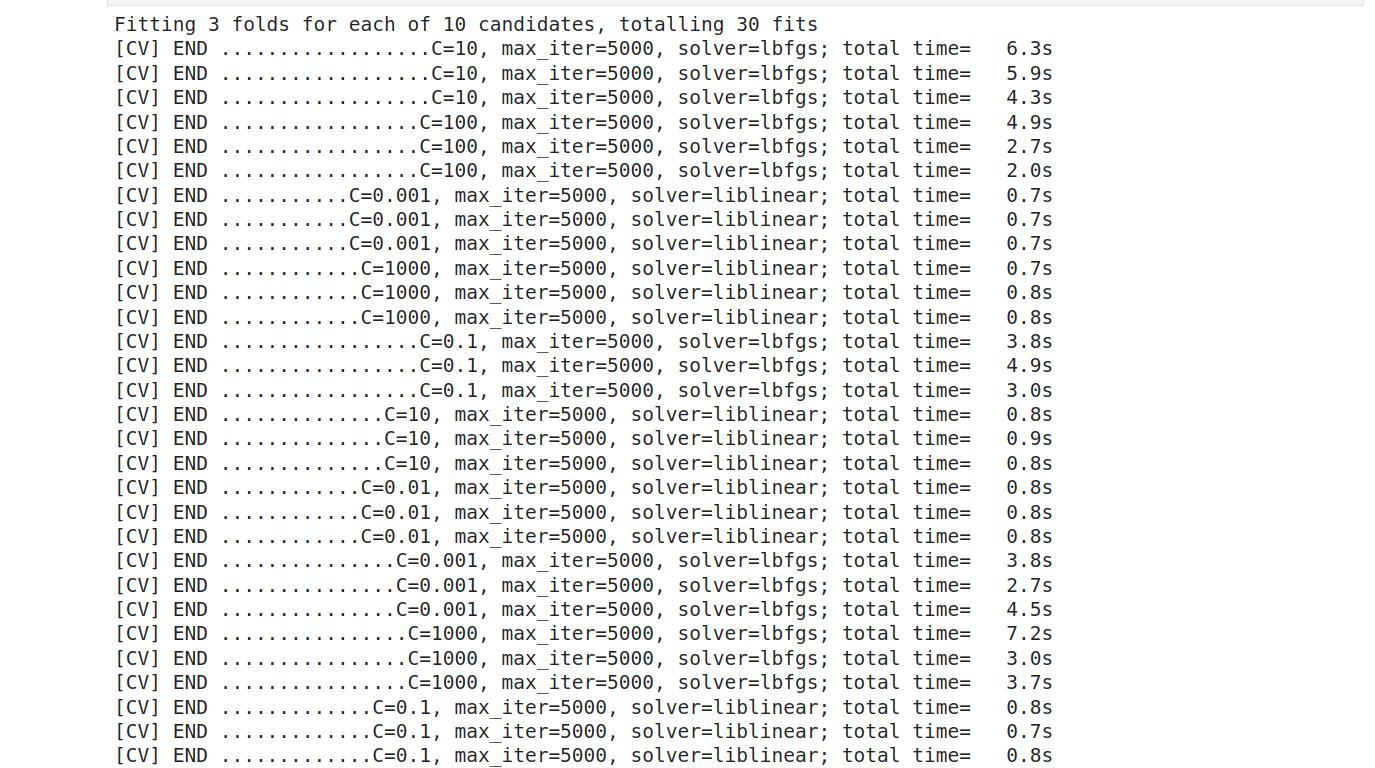Find the best parameters and predict on evaluation data.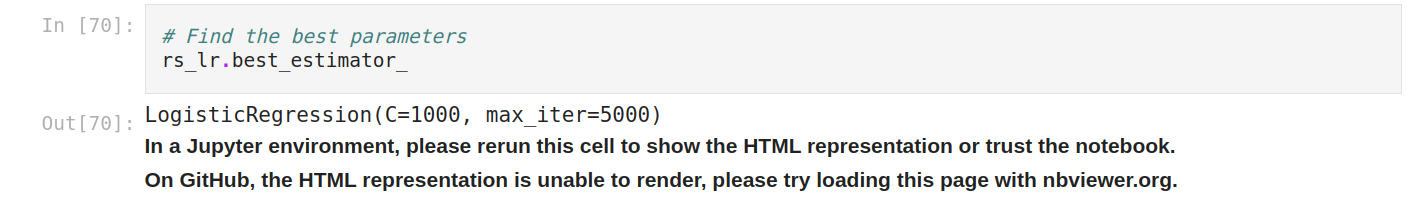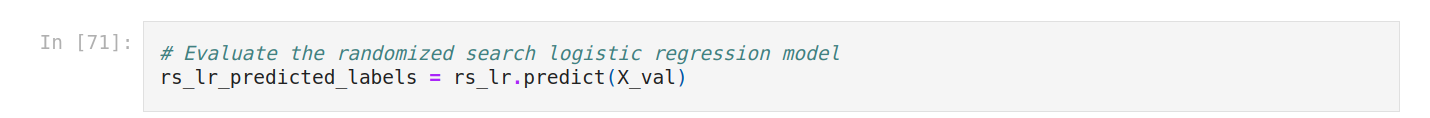Then plot the result.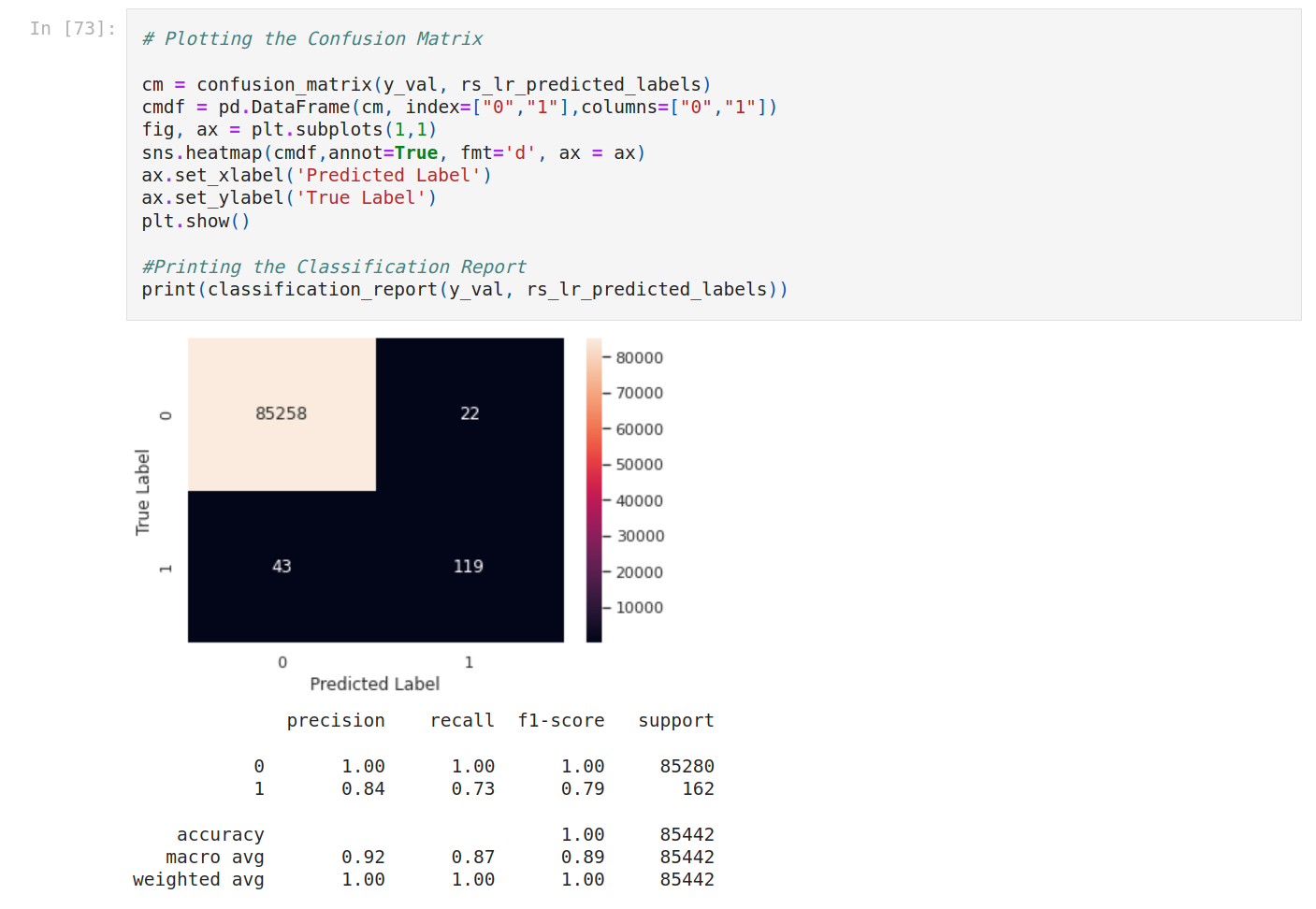We do the same for random forest with GridSearchCV.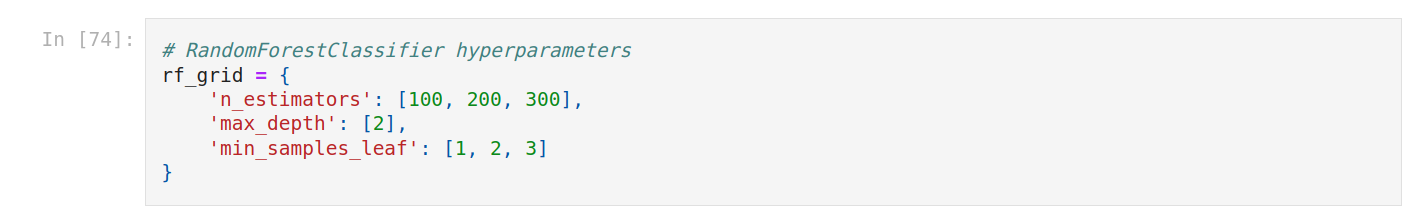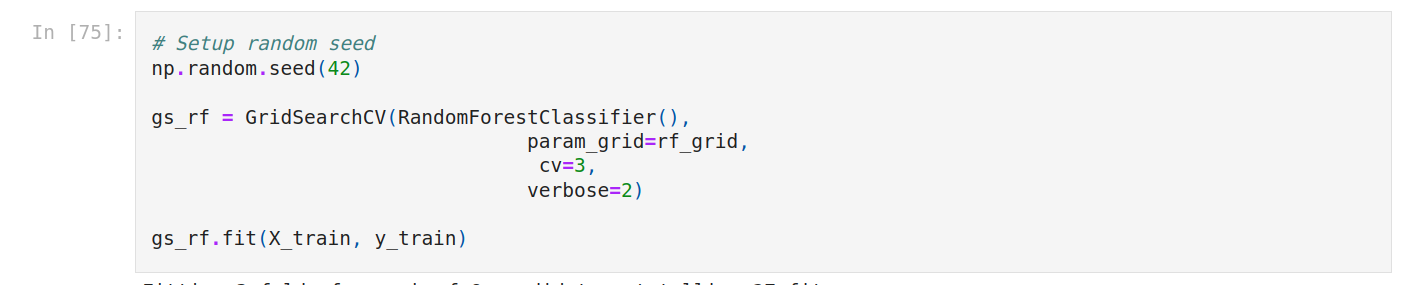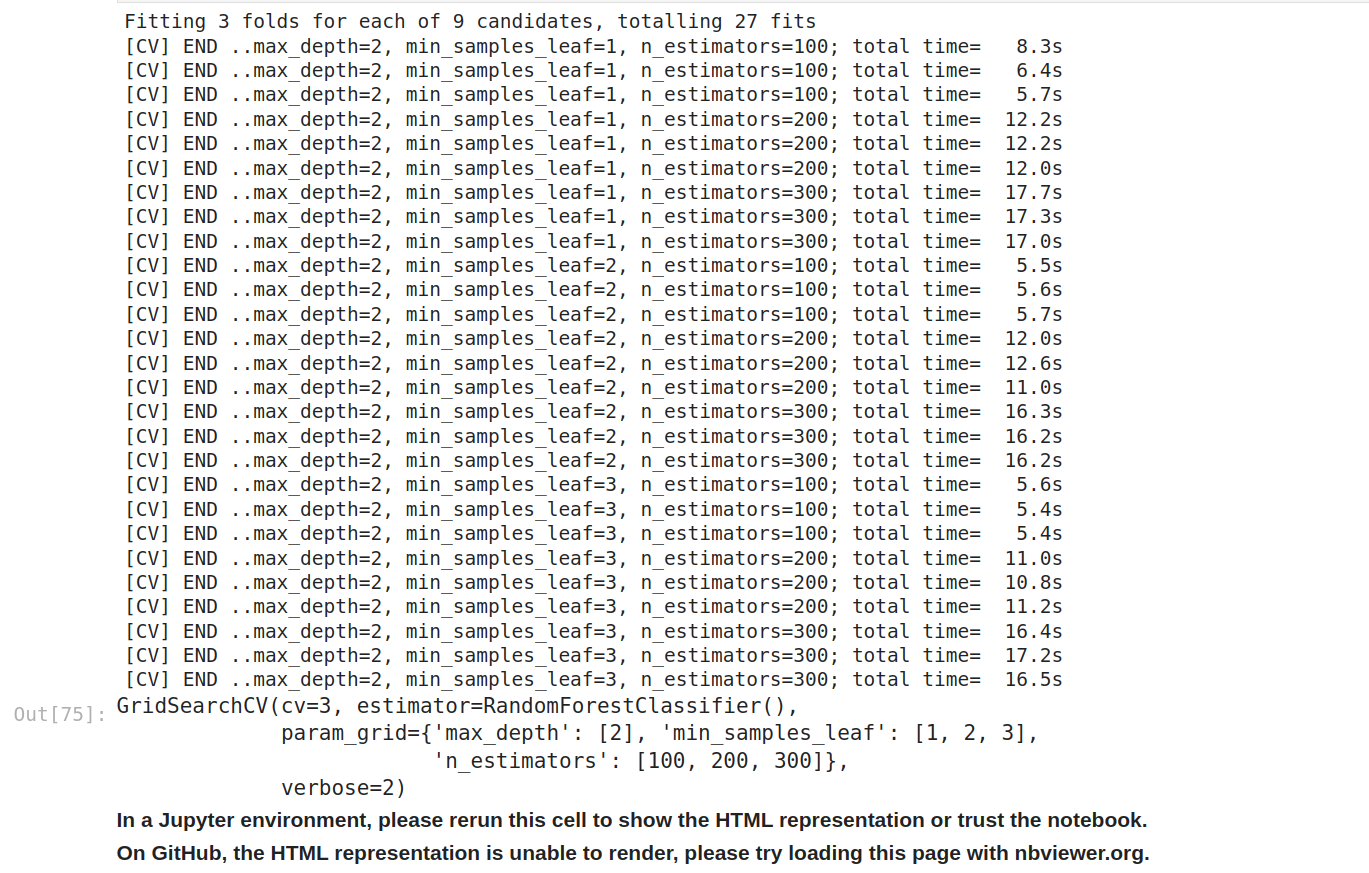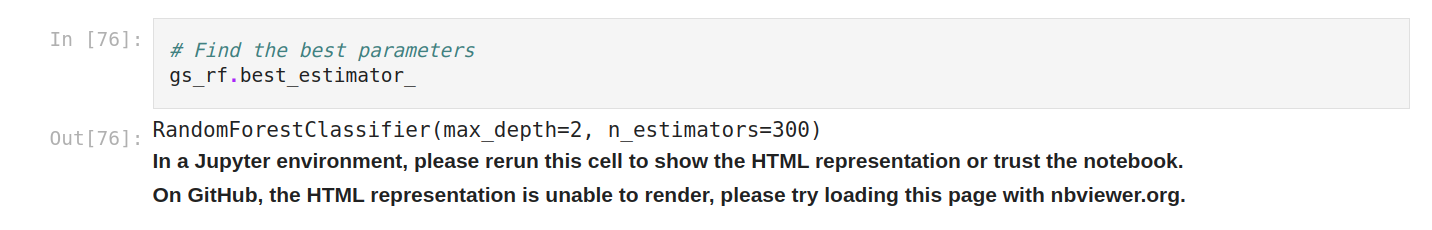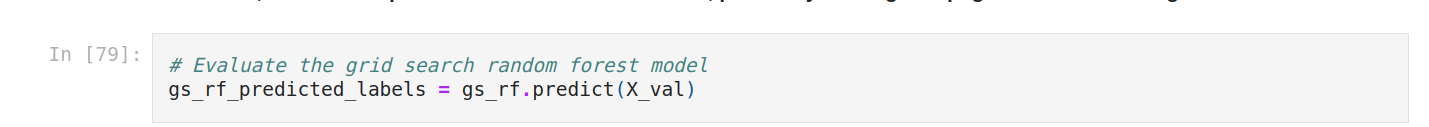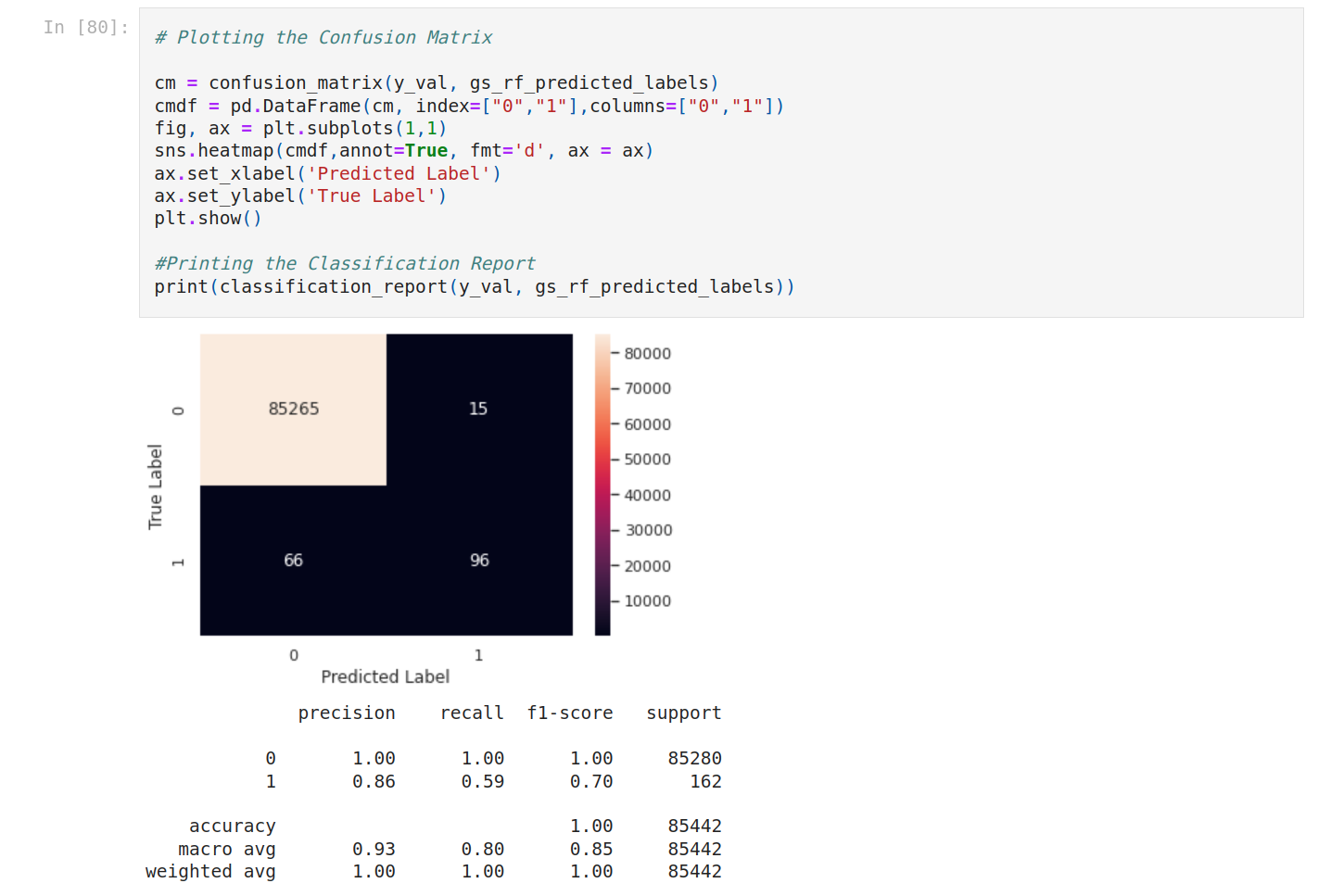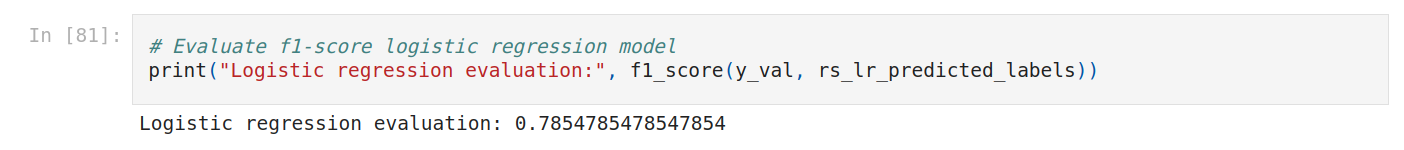We compare F1 scores.It’s better for logistic regression but worse for random forest. It could be complicated to find the best grid. The problem is that it takes time to process. So we stop here.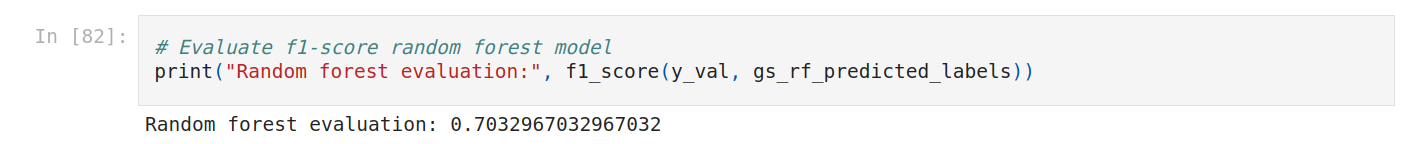Let’s plot ROC curve.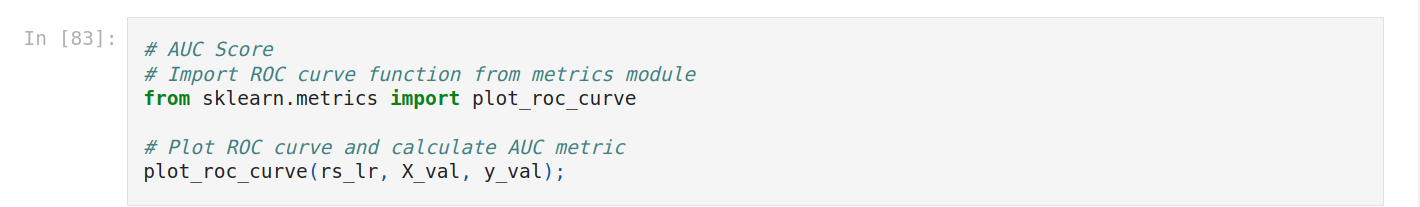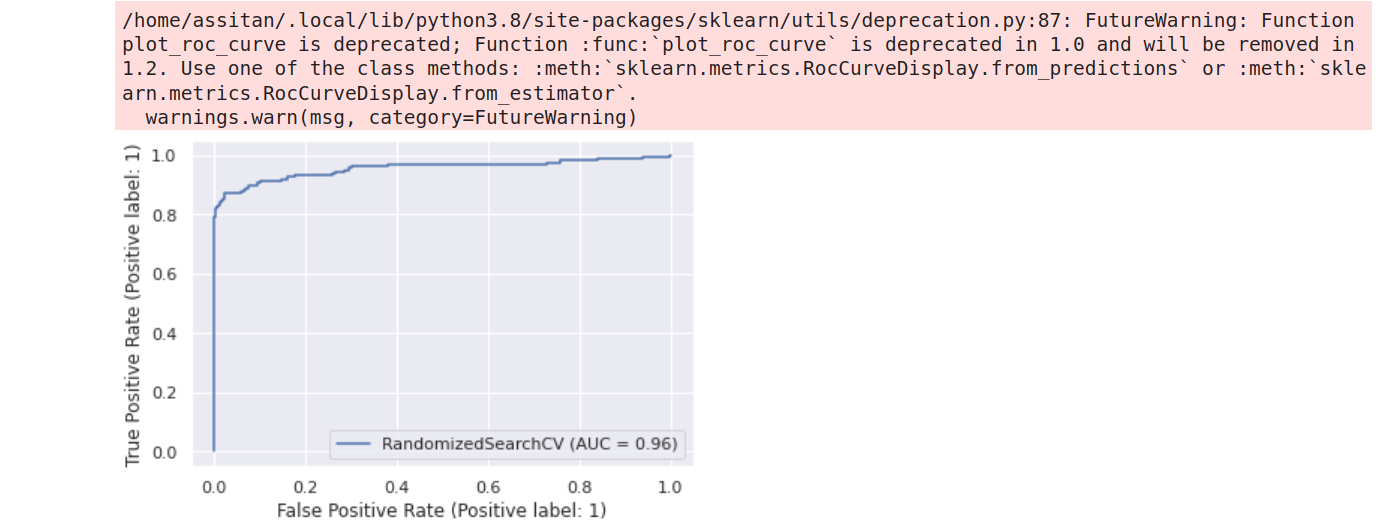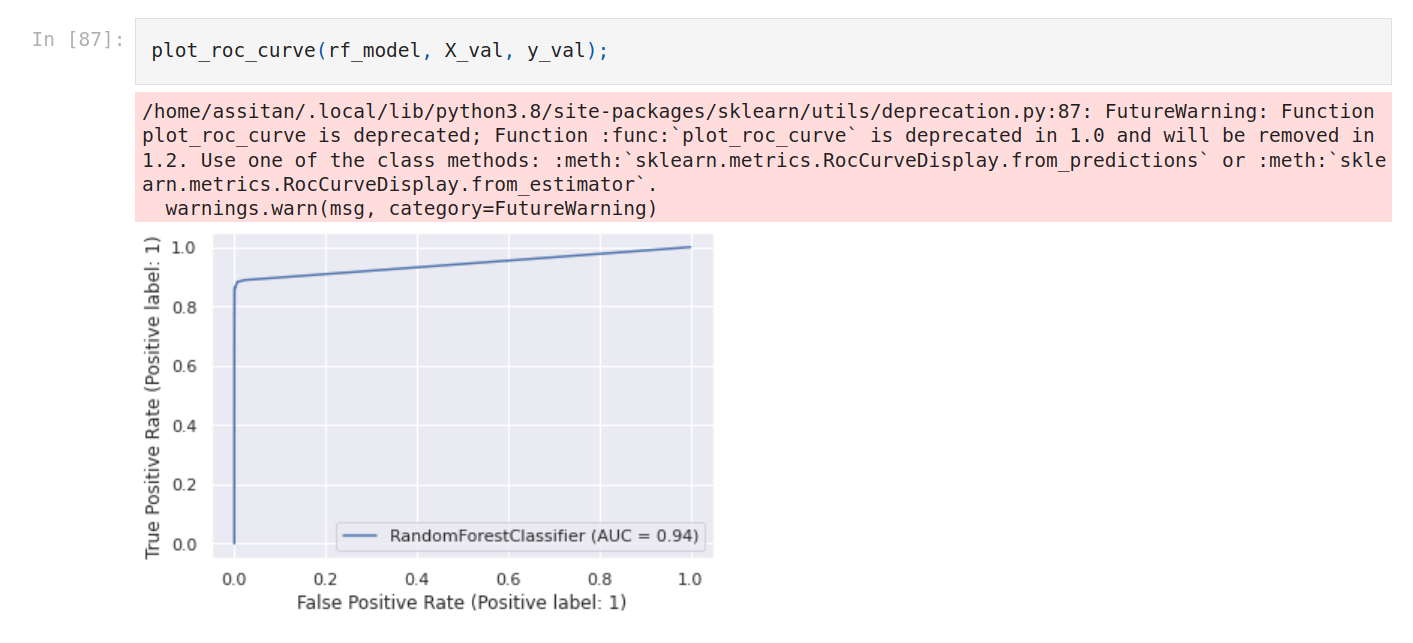As seen above the area under the ROC curve varies from 0 to 1, with 1 being ideal and 0.5 being random. An AUC (Area Under the ROC Curve) of 0.9 is indicative of a reasonably good model; 0.8 is alright, 0.7 is not very good, and 0.6 indicates quite poor performance. The score is indicative of how good the model separates positive and negative labels.

Logistic regression is better. We’re gonna use the first model with Random forest.

### 8. Testing the model

Now let’s test the model. We do the same as evaluation.

We make predictions on test data (probability).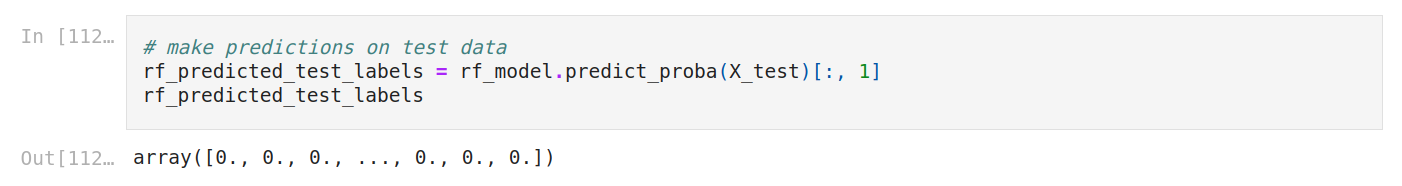The output is a matrix with predictions. For each credit card, it outputs two numbers, which are the probability of being non fraudulent and the probability of being fraudulent. We select the second column, we don't need both (the probability of being fraudulent).

Let’s use the model. We’re gonna select a credit card from the test data. Let’s display it as a dict so you see the data.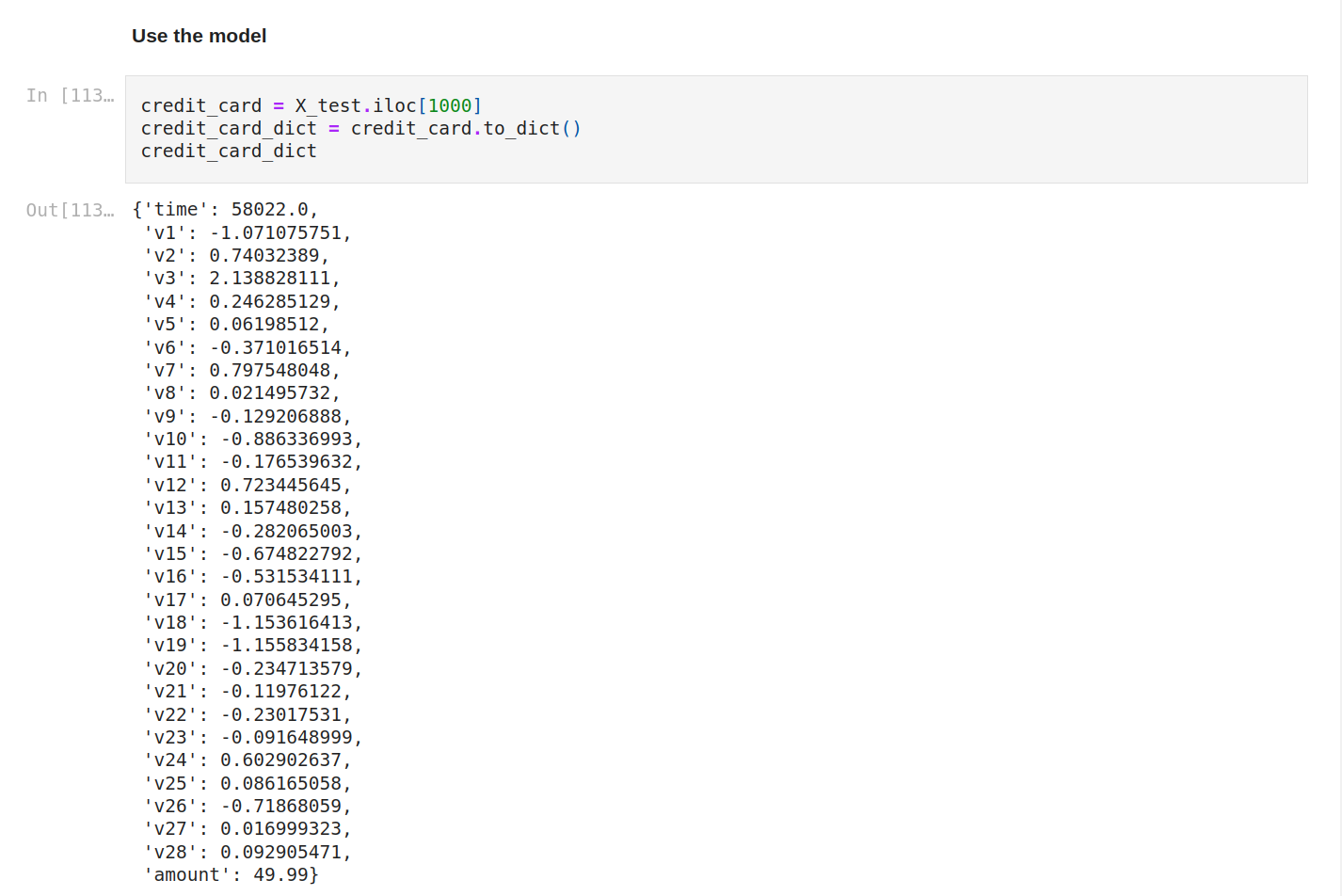Now we make a prediction on this credit card. This time, there's just one row and we get the second column so we set just zero.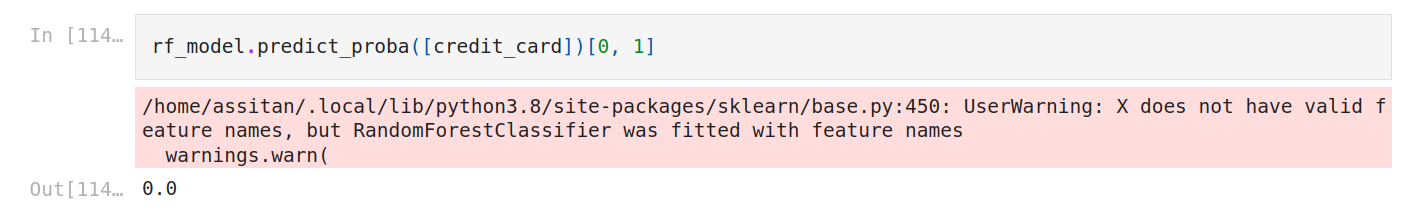We get zero.

Let’s check the result.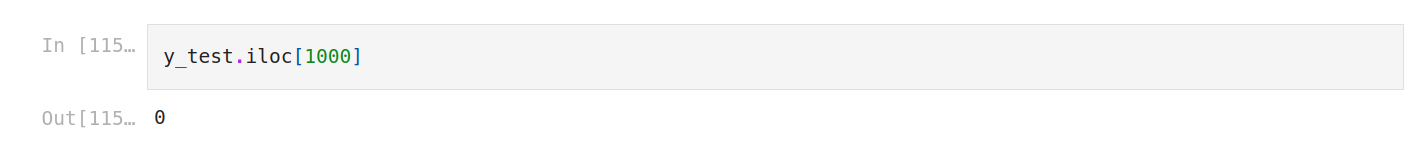Also zero. So our model had guessed well.

That's it.

What you can do now is deploy the model or improve the score. To improve the score, you can do some feature engineering or try other combinations for the hyperparameters.

See you next time for the second part.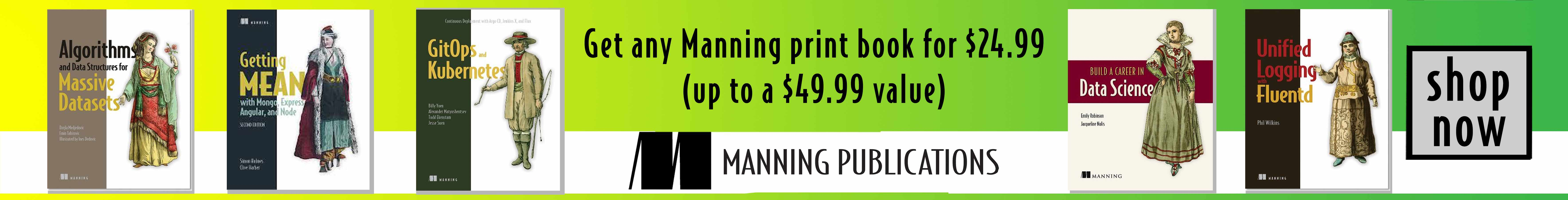### Resources

Écrit parAssitan Koné

Software engineer senior | ML Engineer. Also a digital arts graduate, I love explaining data science and programming concepts with illustrations.

#### Inscrivez-vous. Soyez inspiré.e. Codez.

Tutoriels, illustrations et conseils dans votre boite email.